# RD Sharma Solutions for Class 10 Maths Chapter 15 Areas Related To Circles

## RD Sharma Solutions Class 10 Maths Chapter 15 – Avail Free PDF (for 2021 – 22)

RD Sharma Solutions for Class 10 Maths Chapter 15 – Areas Related to Circles is given here for students to enhance their performance in the board examinations. A circle is a very important chapter since we come across many objects related to circular shape in one form or the other in our daily life. Students can make use of the RD Sharma Solutions in depth to know the various methods of solving the problems in an efficient manner.

Chapter 15 – Areas Related To Circles offer precise answers to improve the fundamental concepts among students. For better understanding of concepts, students can refer to RD Sharma Solutions for Class 10 Maths. In this chapter, students learn to solve the problems based on finding the areas of the two special parts of a circular region known as sector and segment of a circle. The main objective of developing the descriptive answers for all the  questions is to provide a best study source for effective exam preparation among students.

## Download the PDF of RD Sharma Solutions For Class 10 Maths Chapter 15 Areas Related To Circles here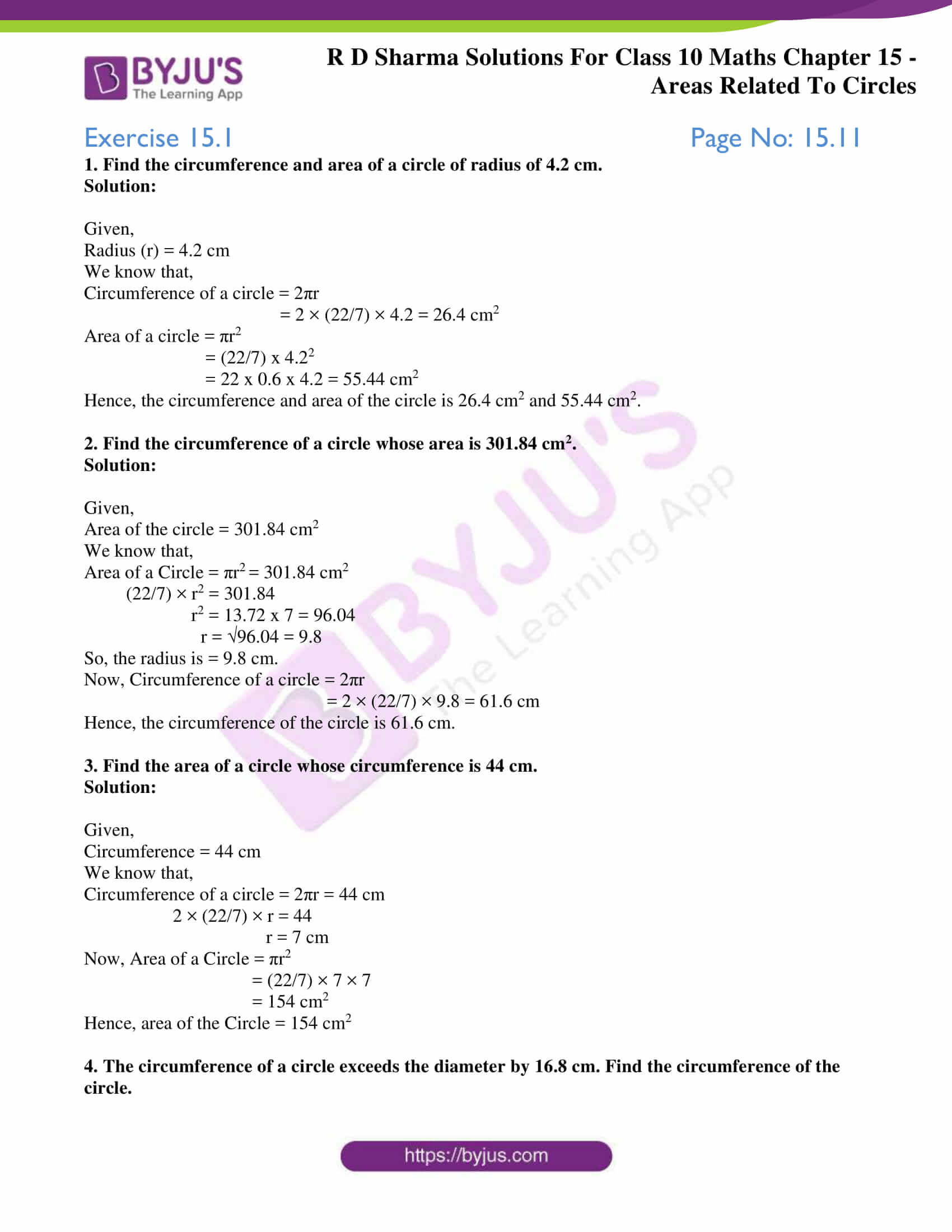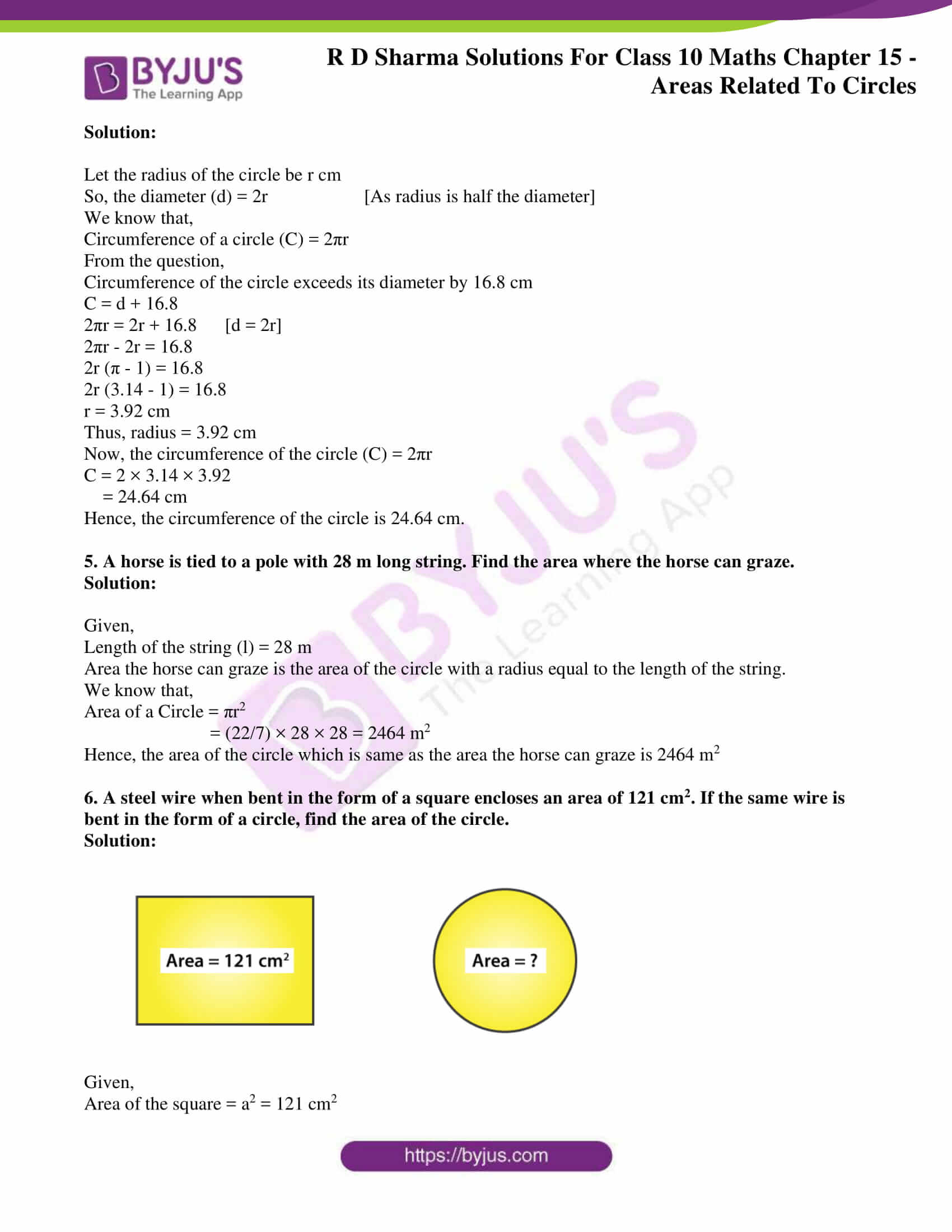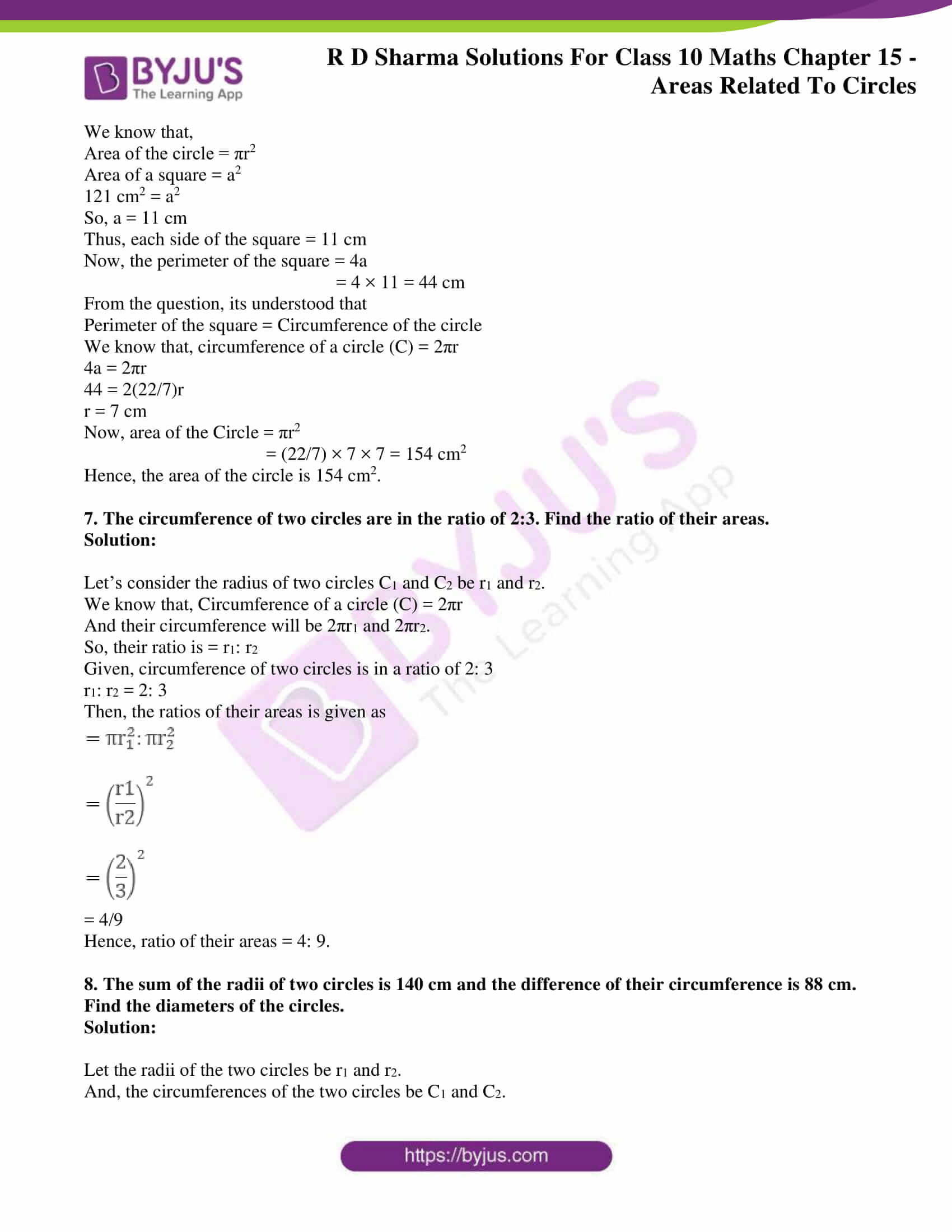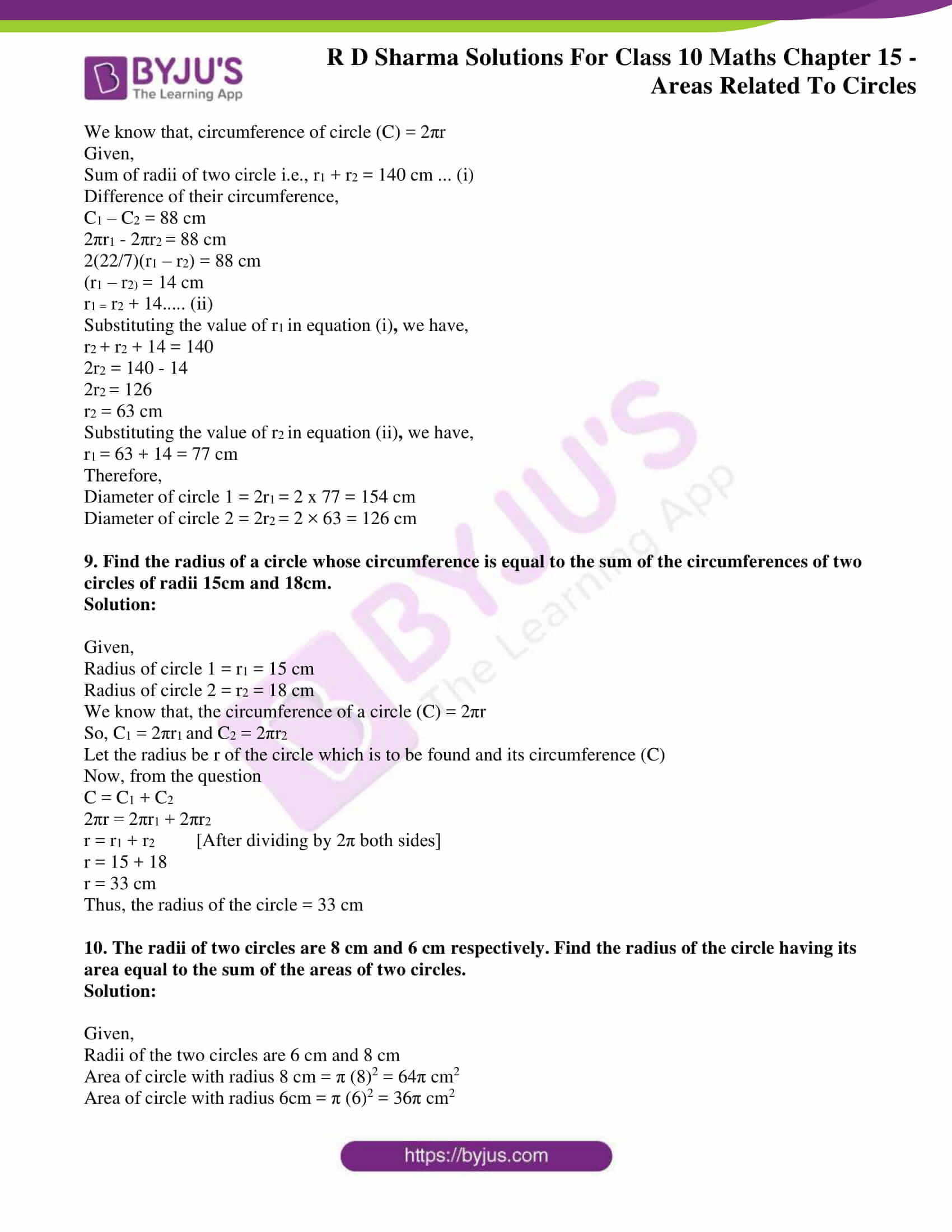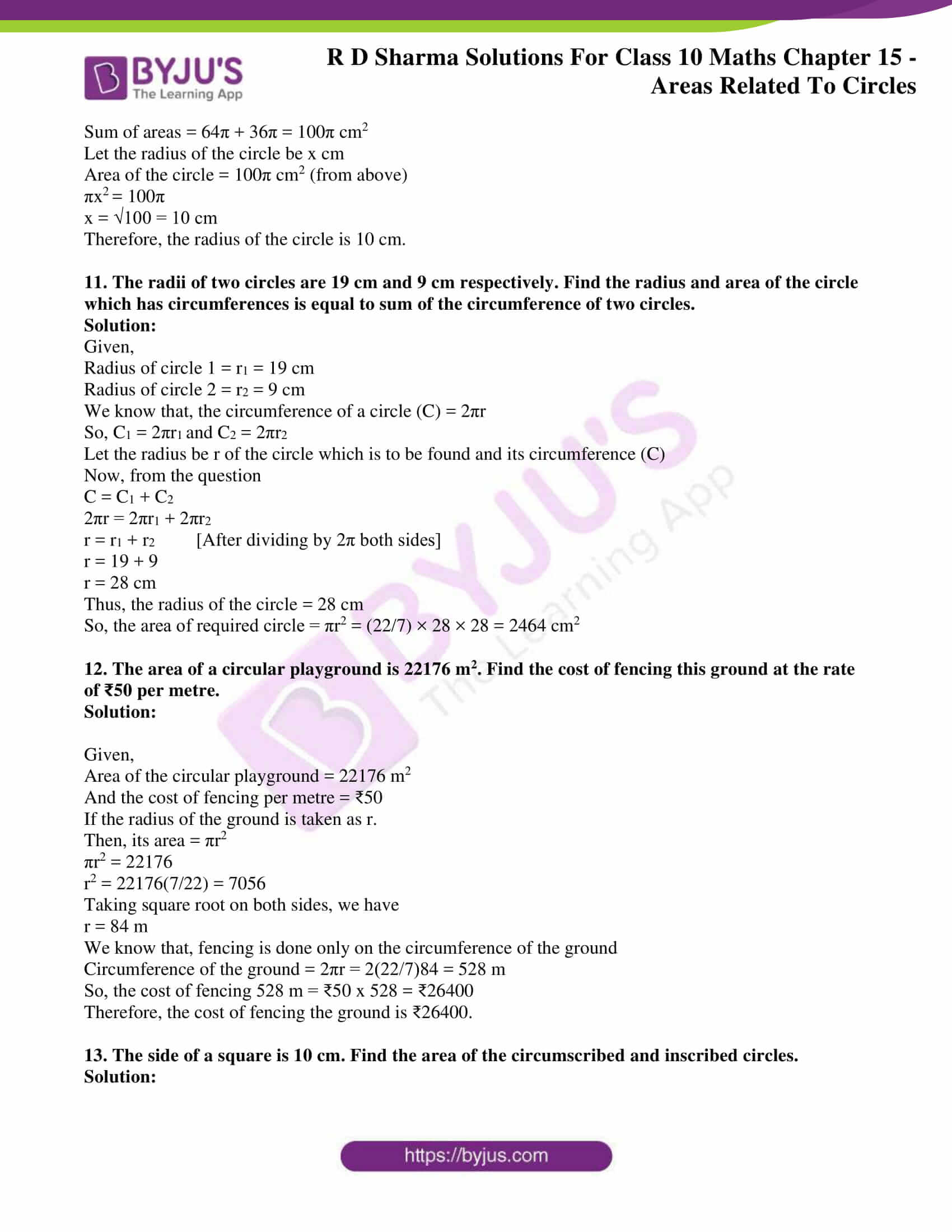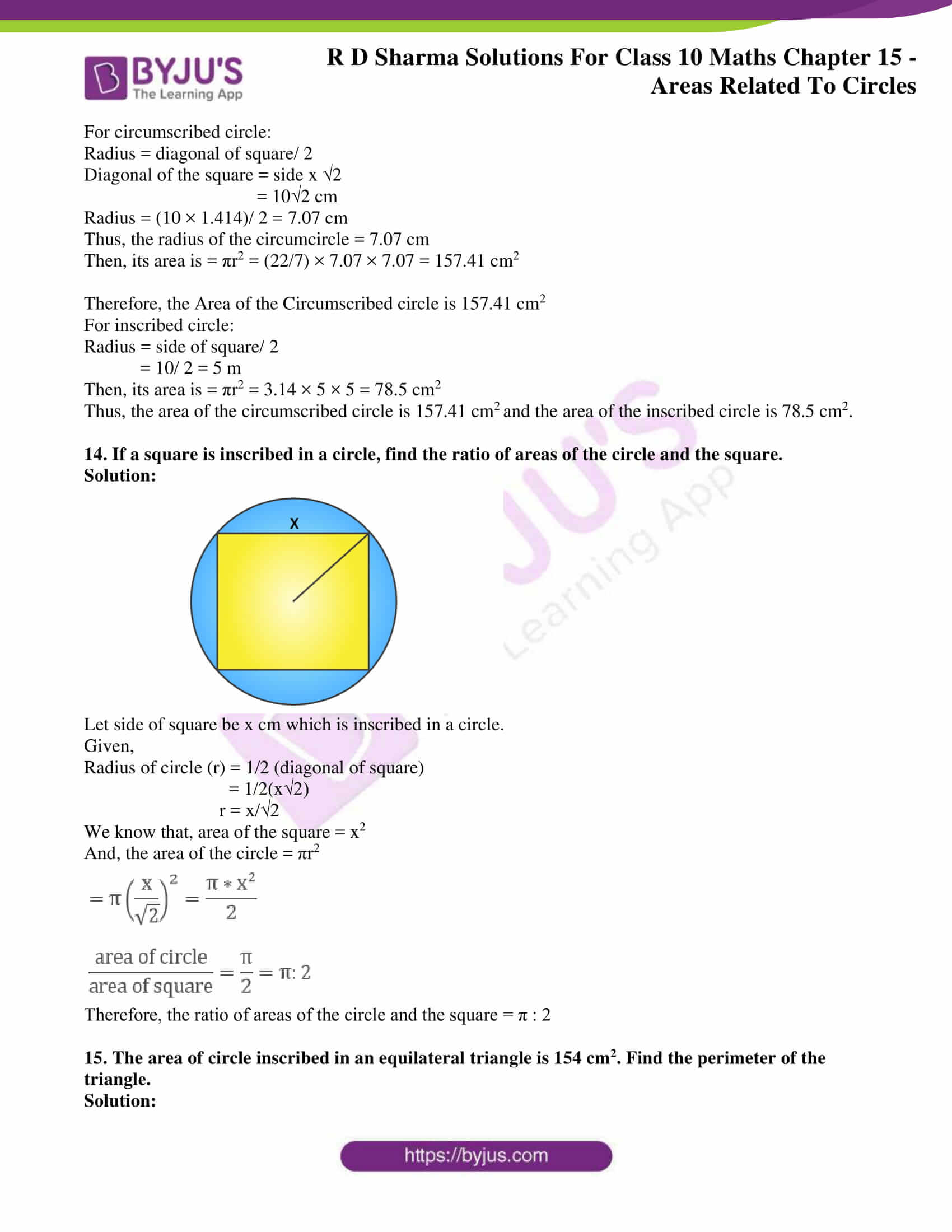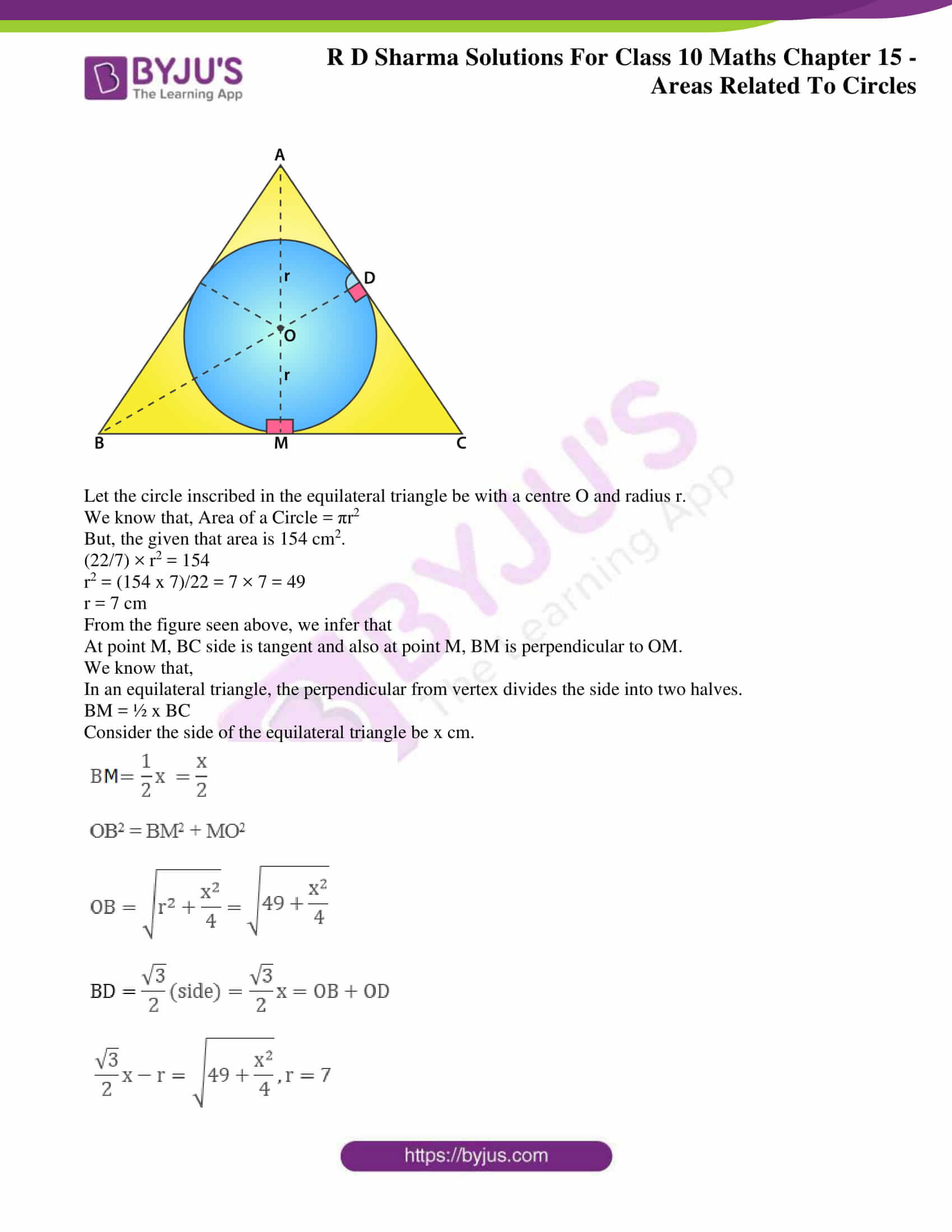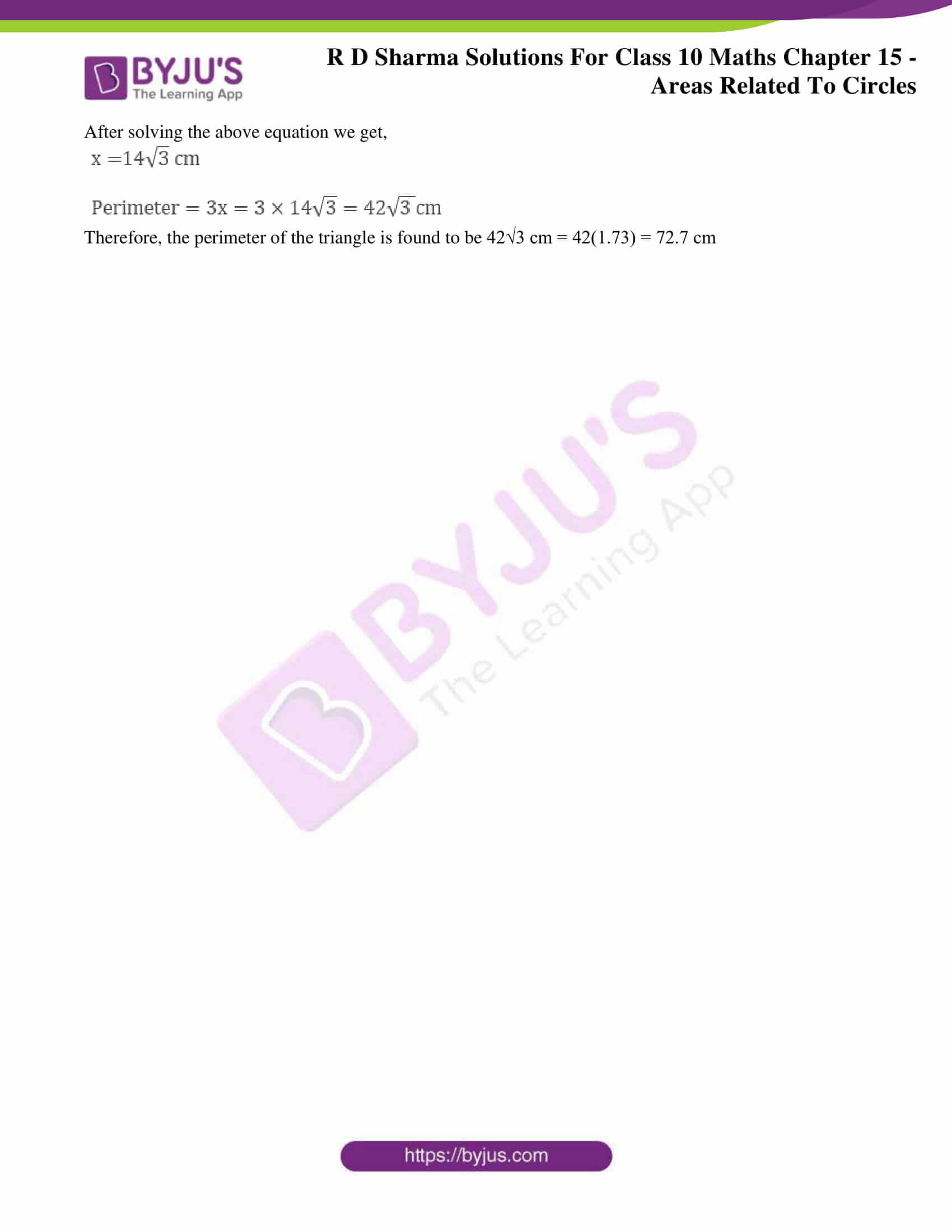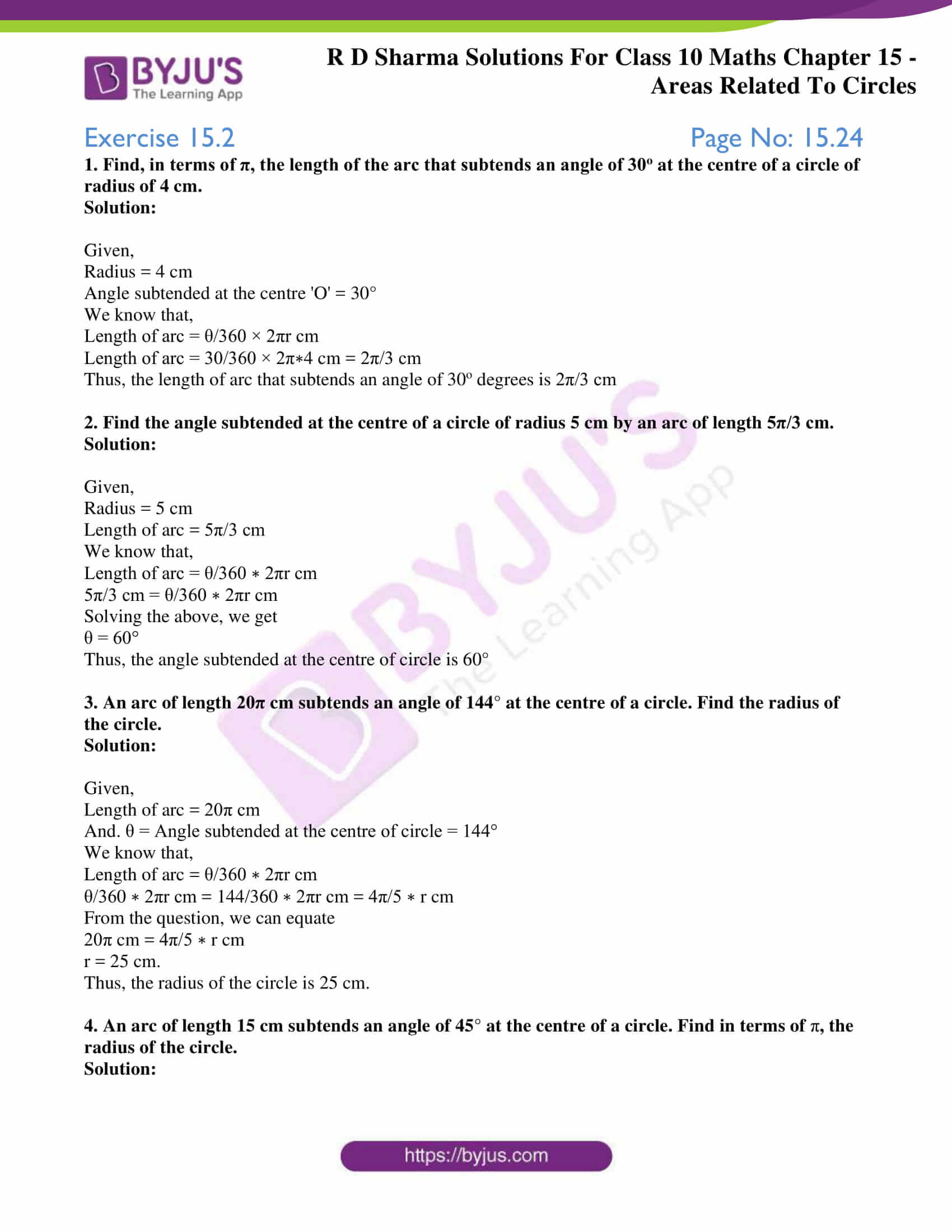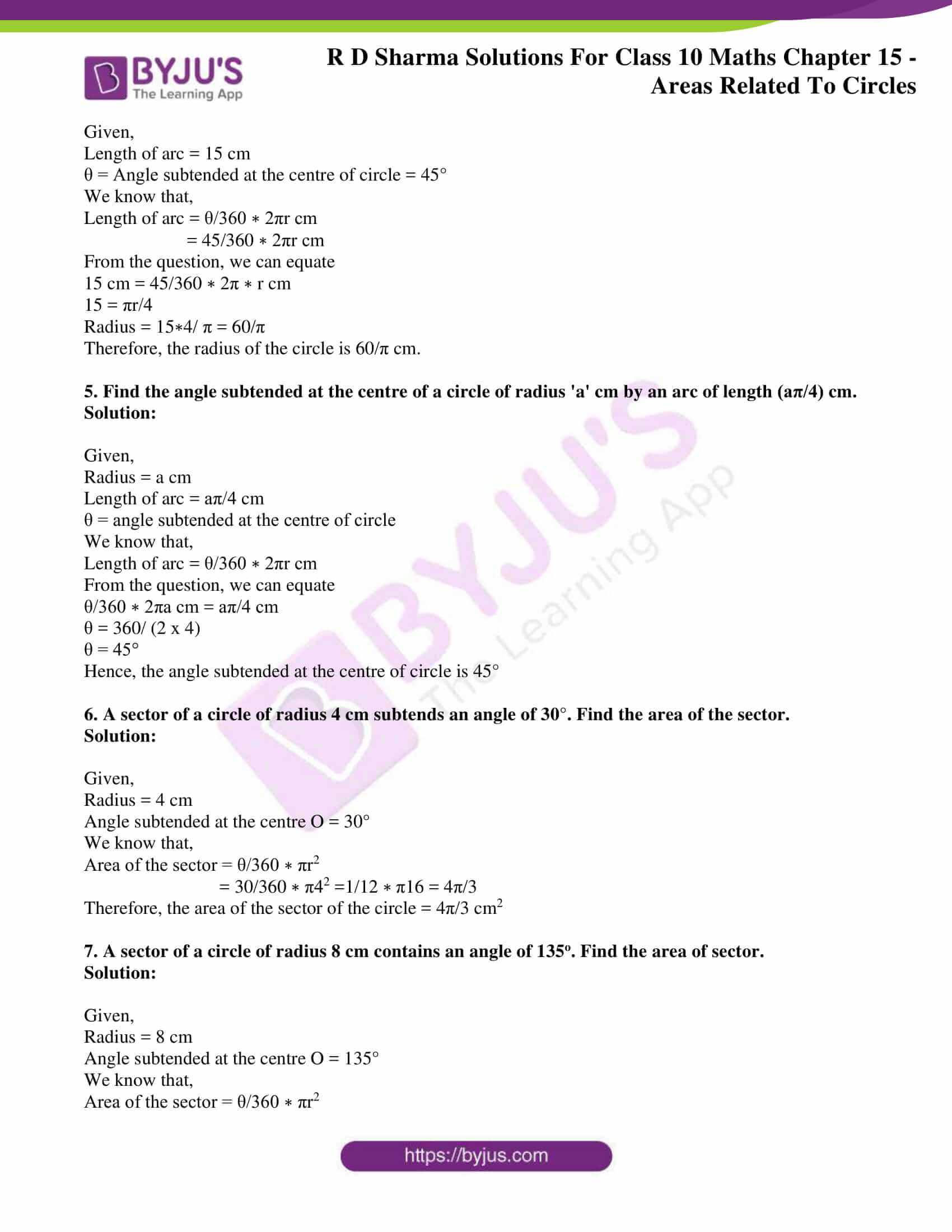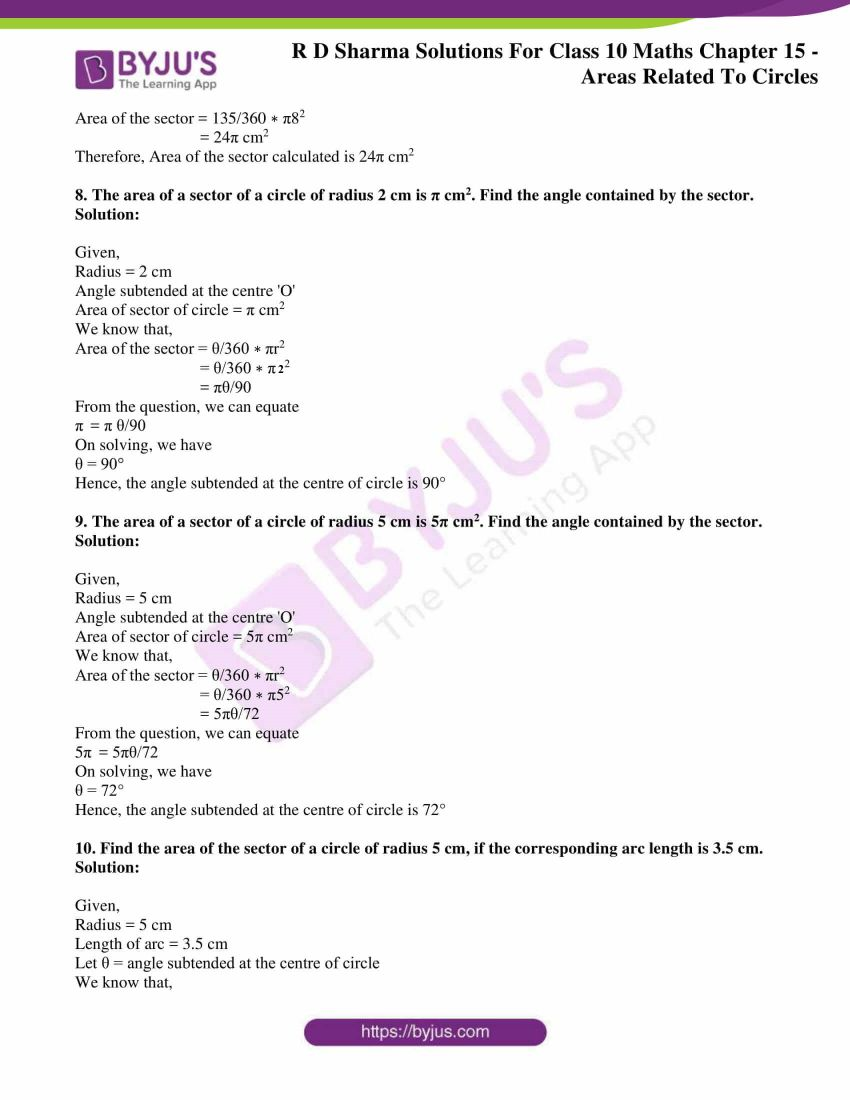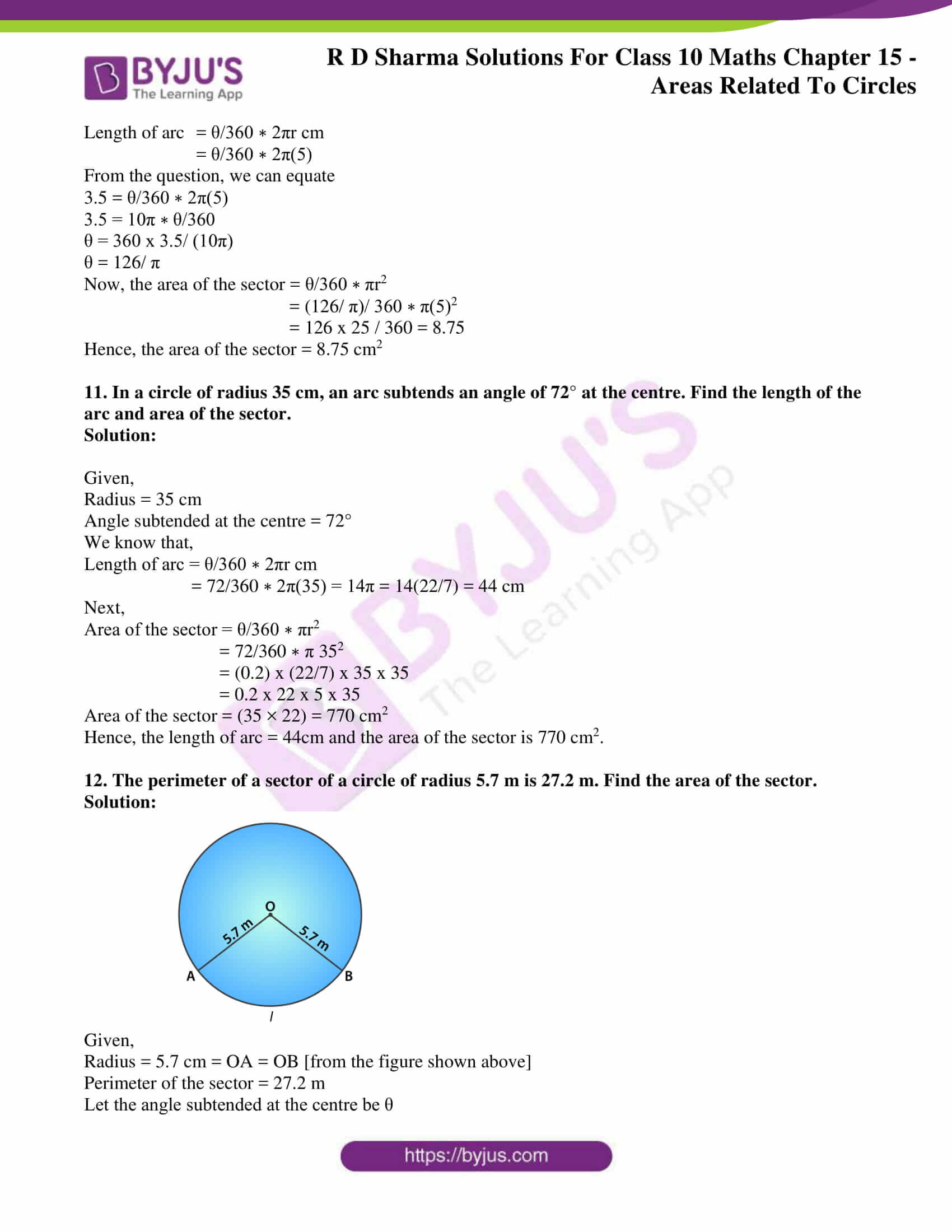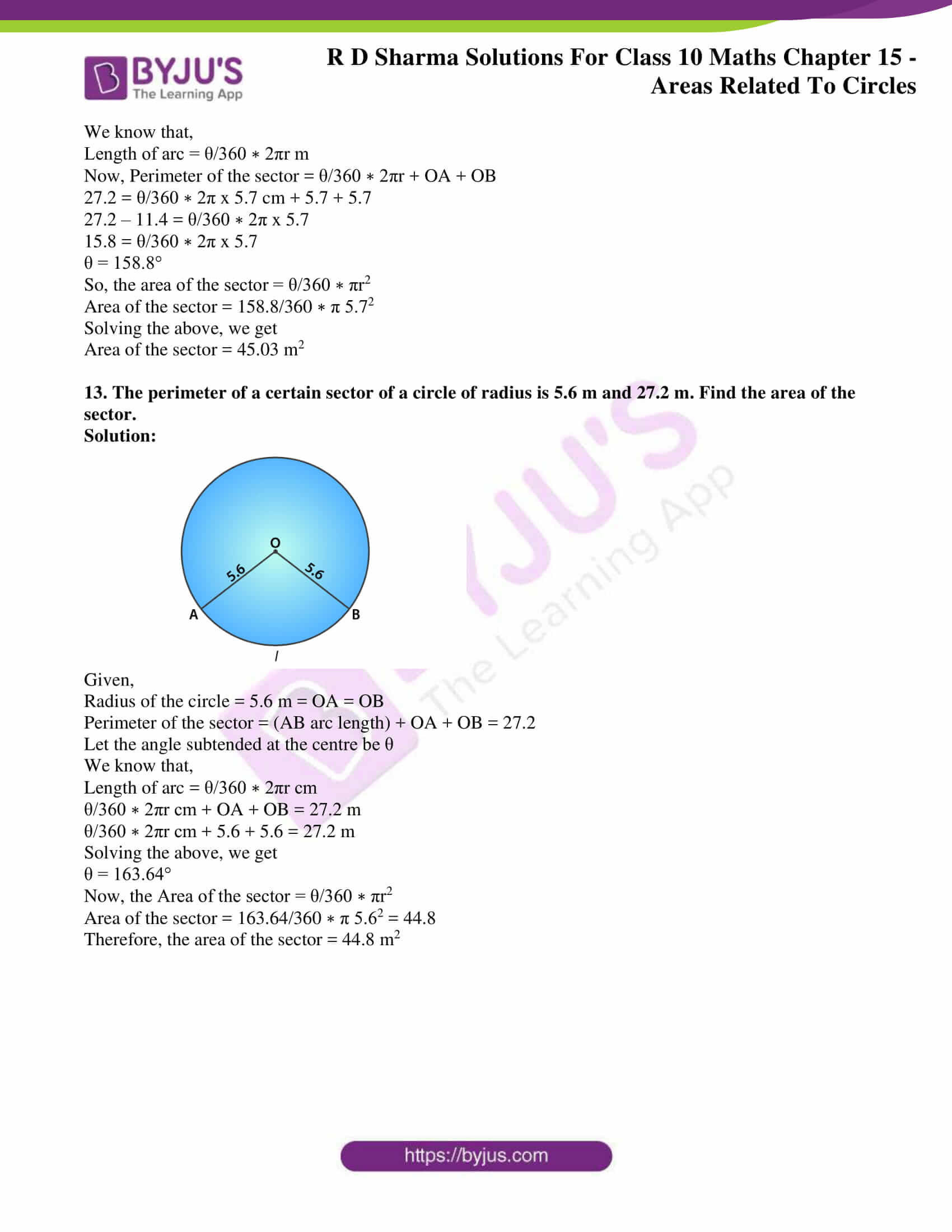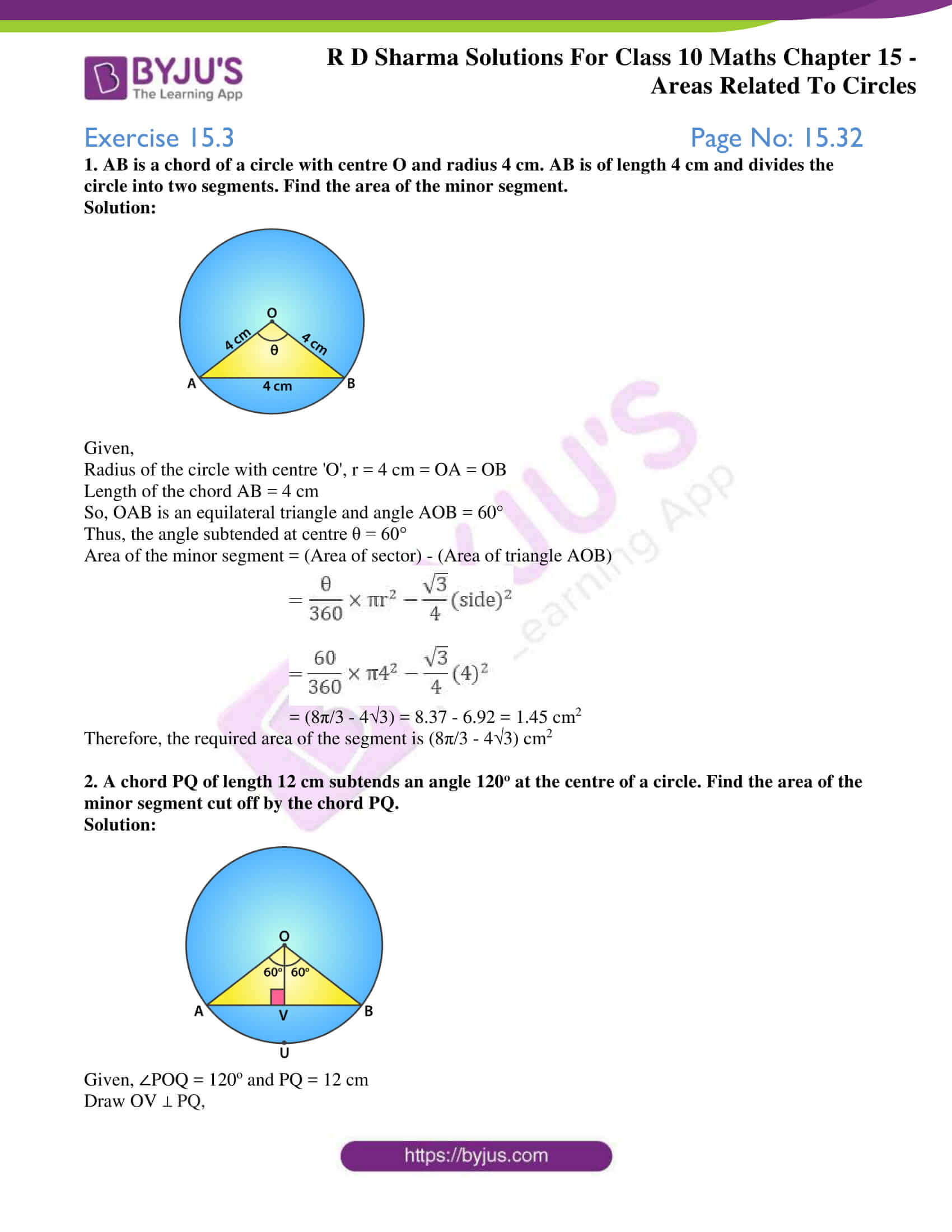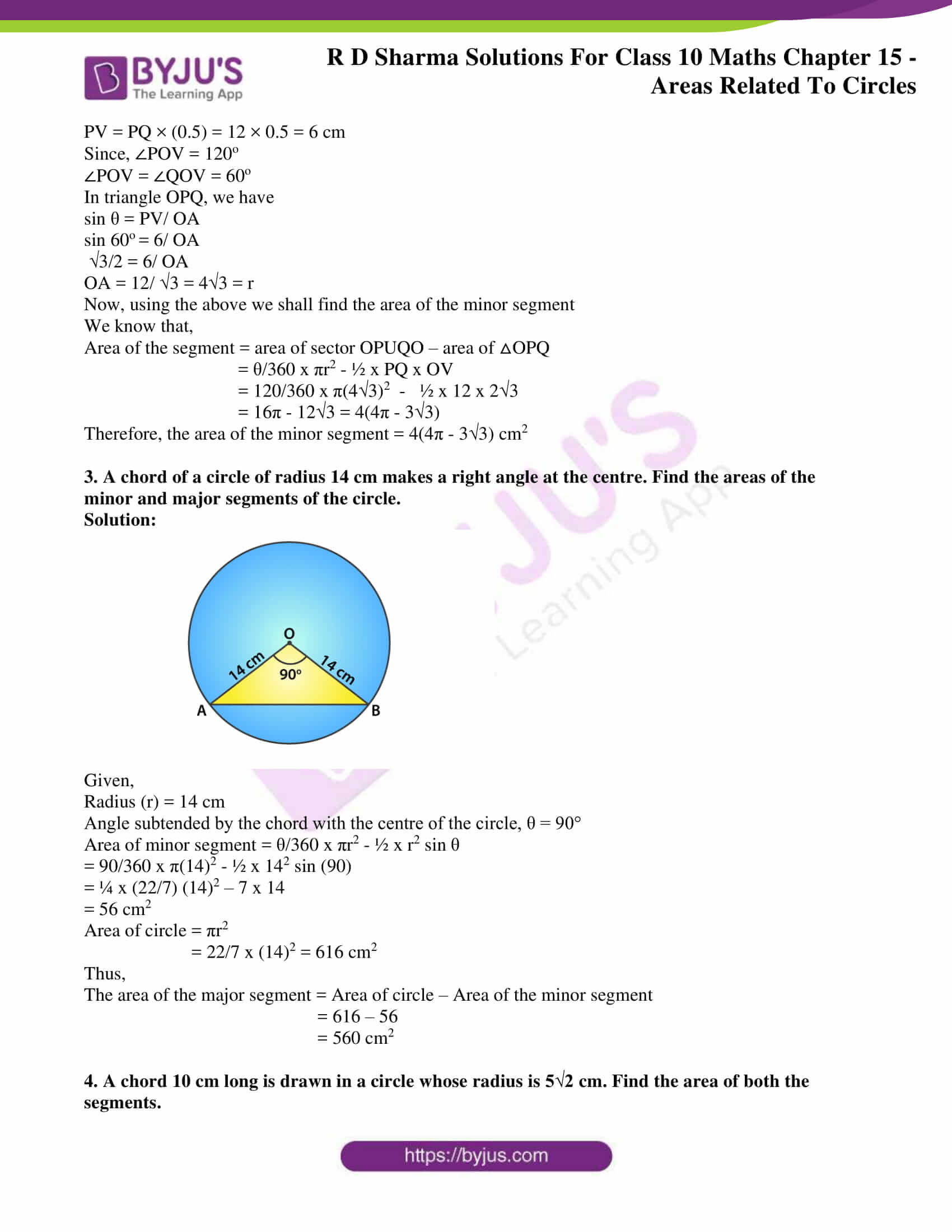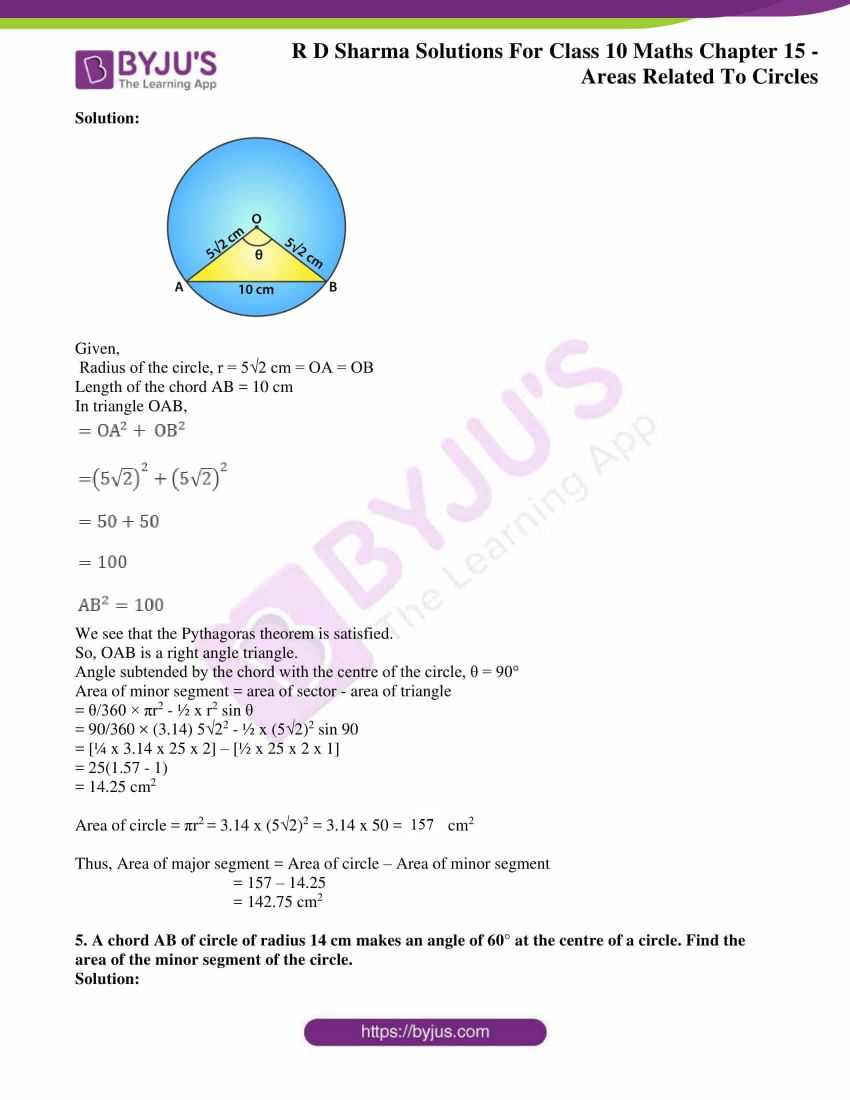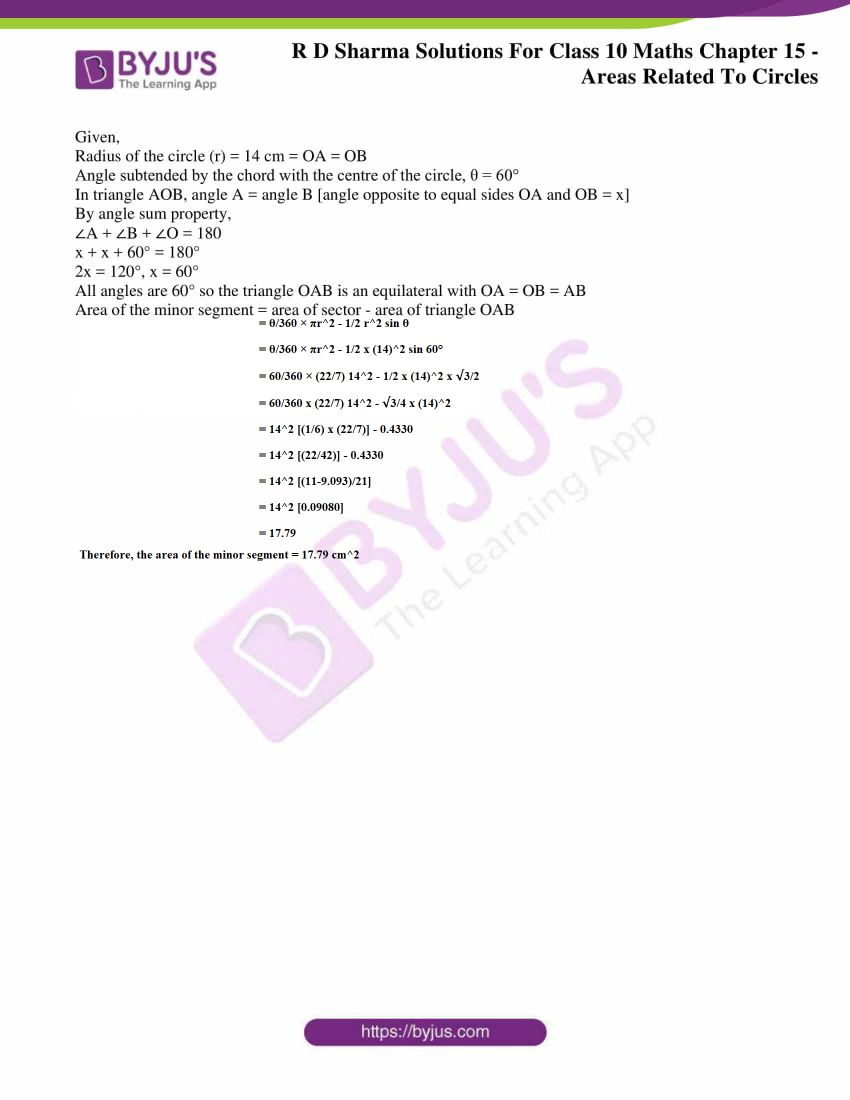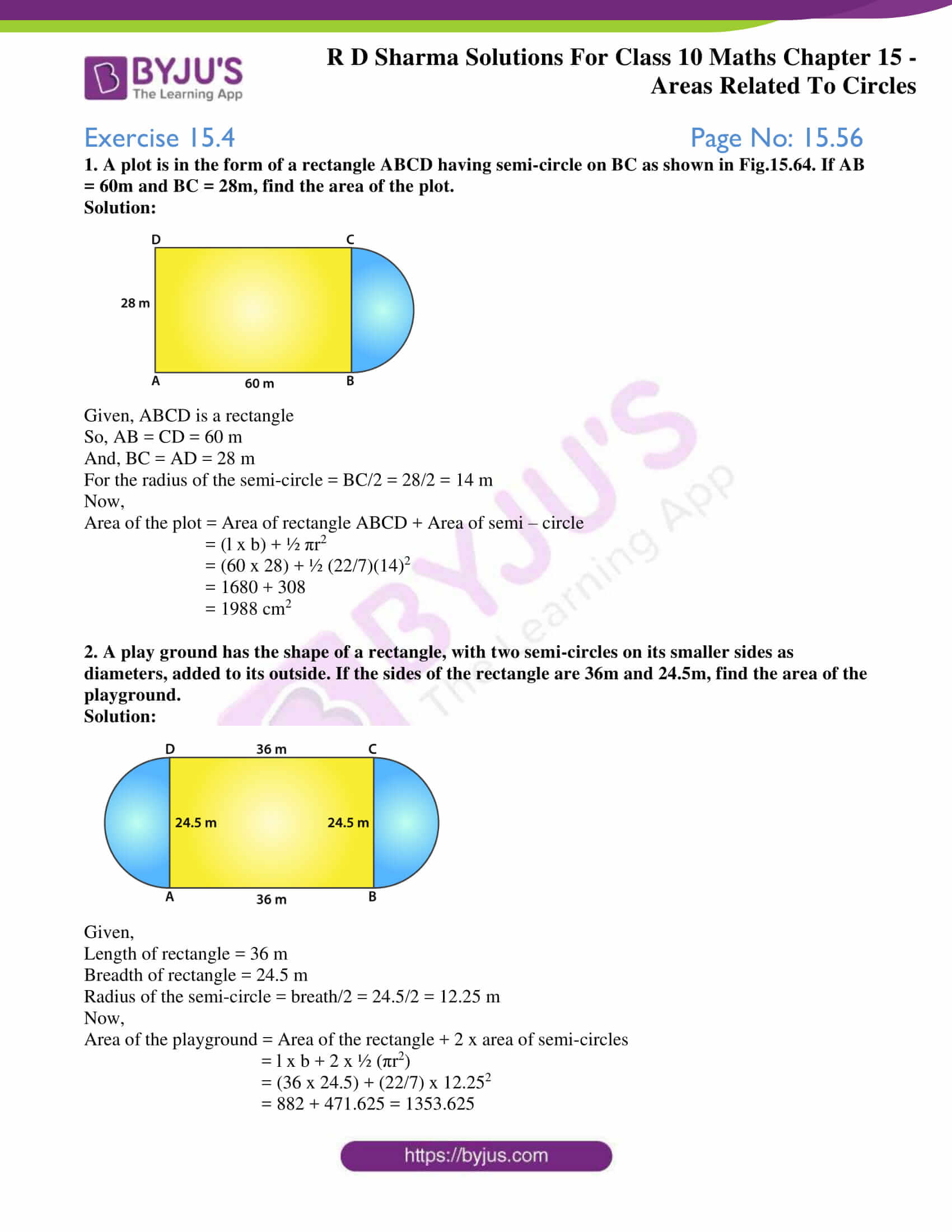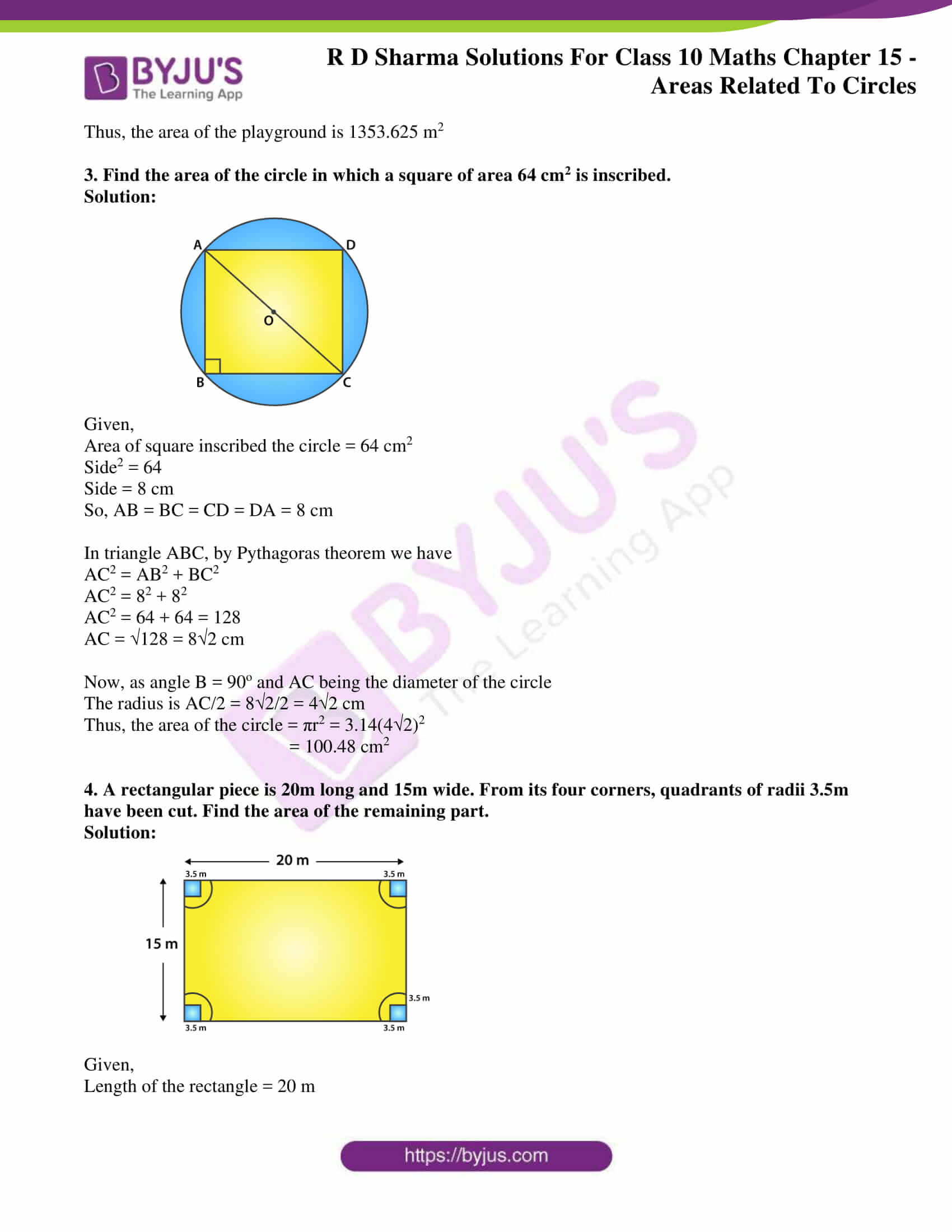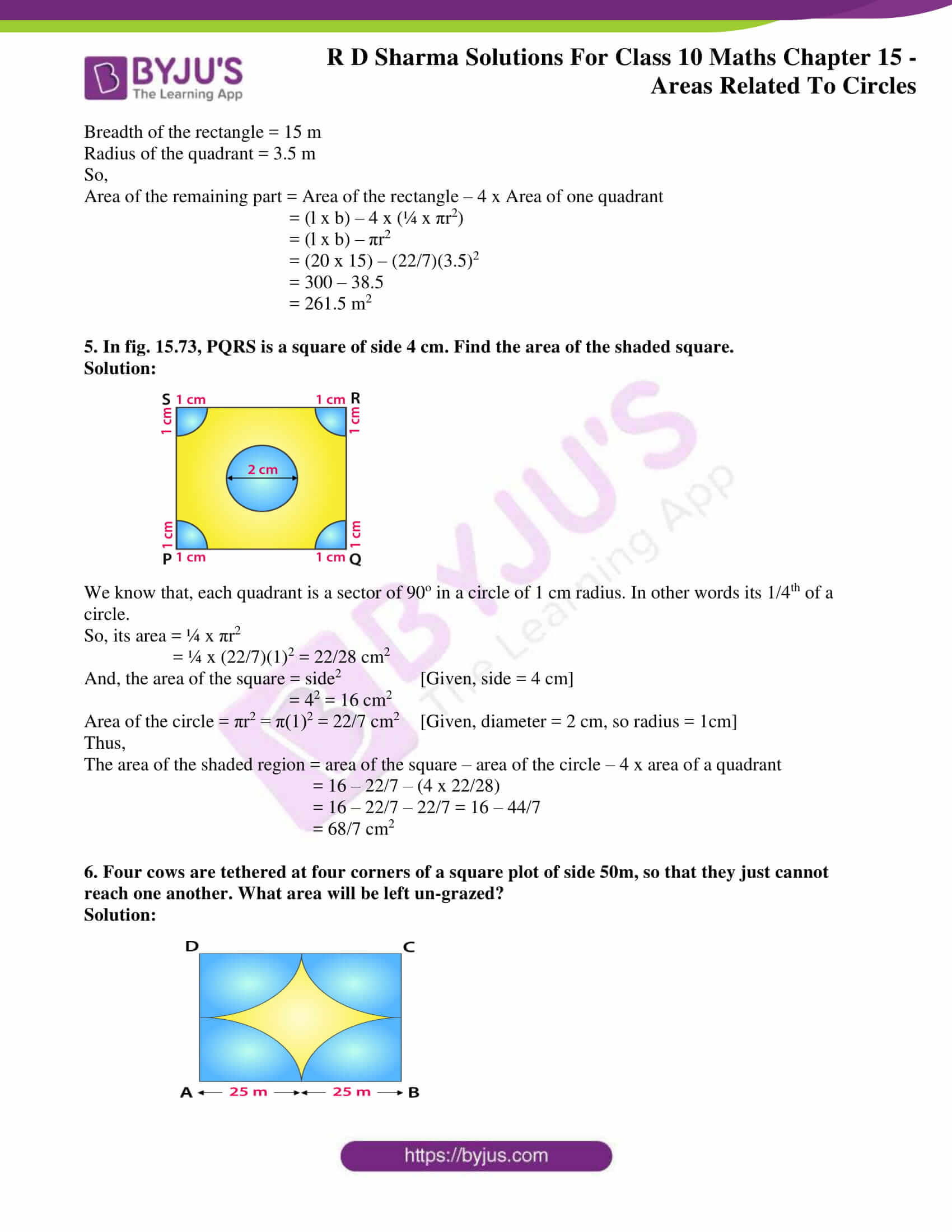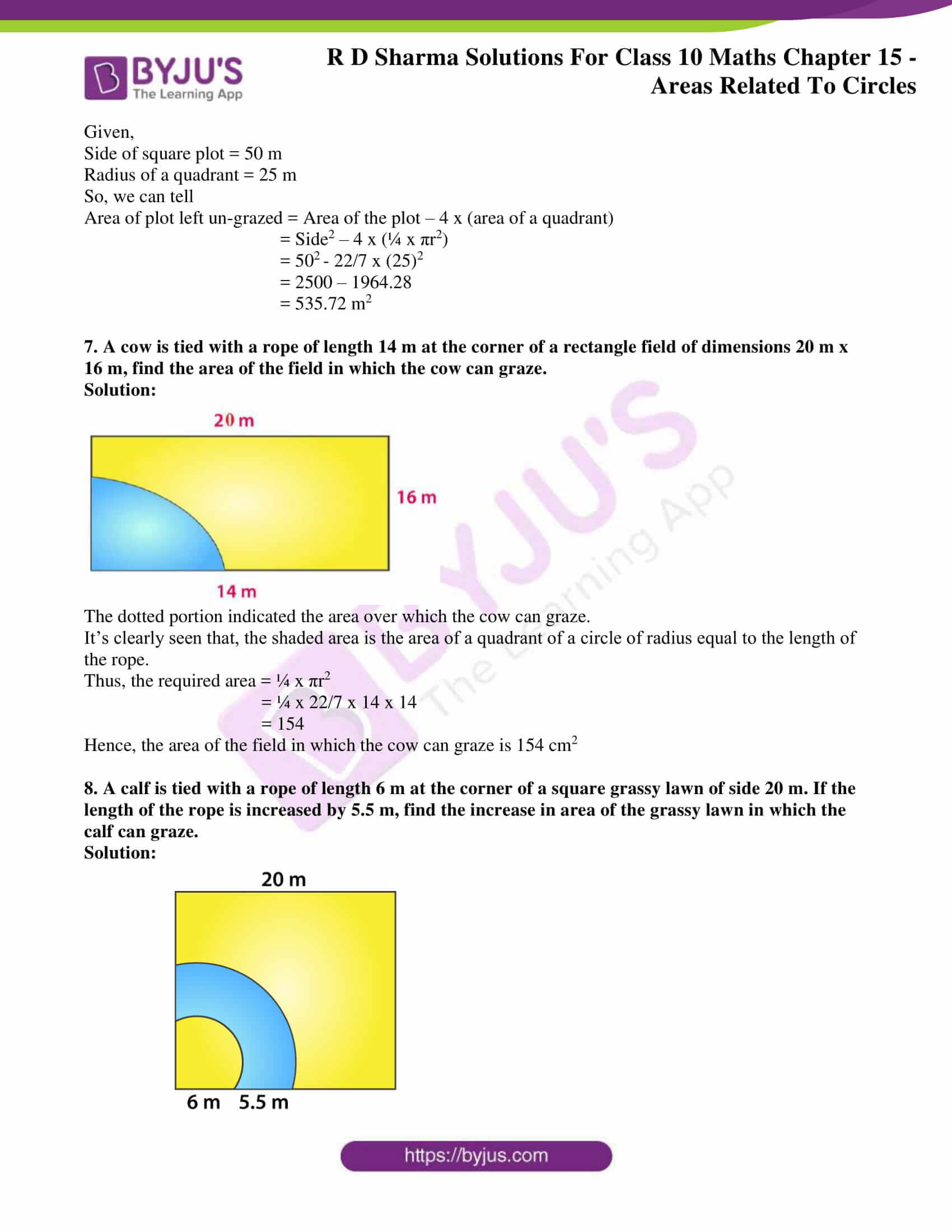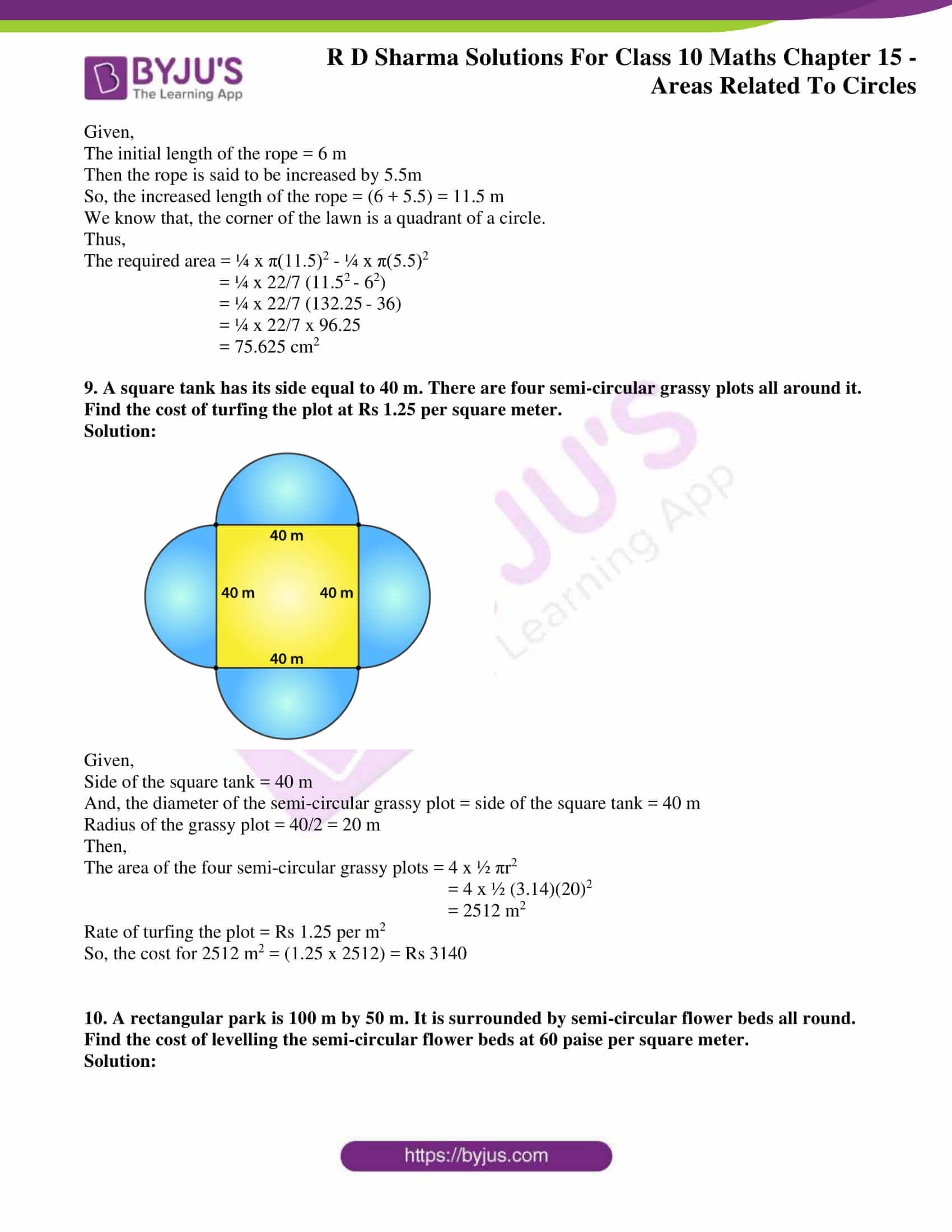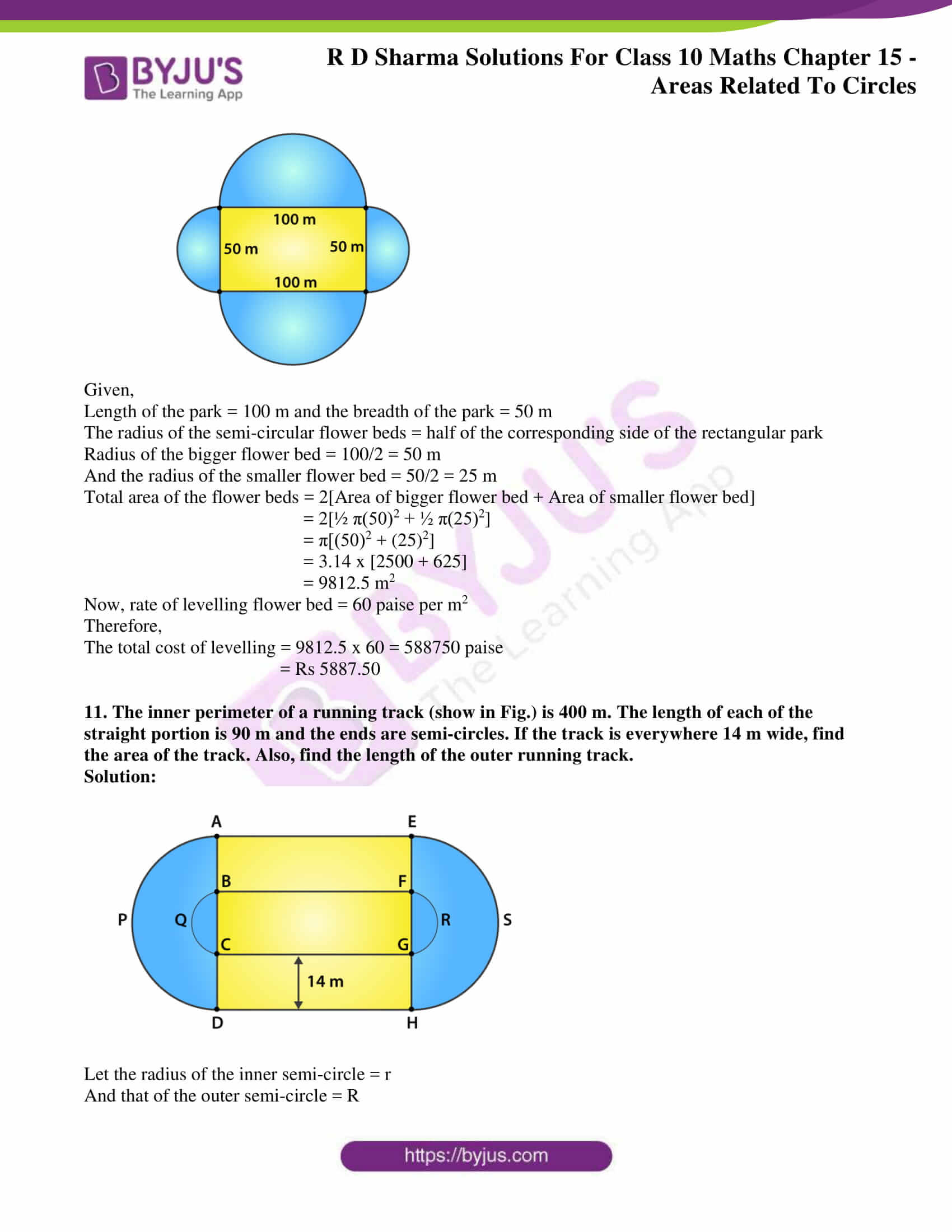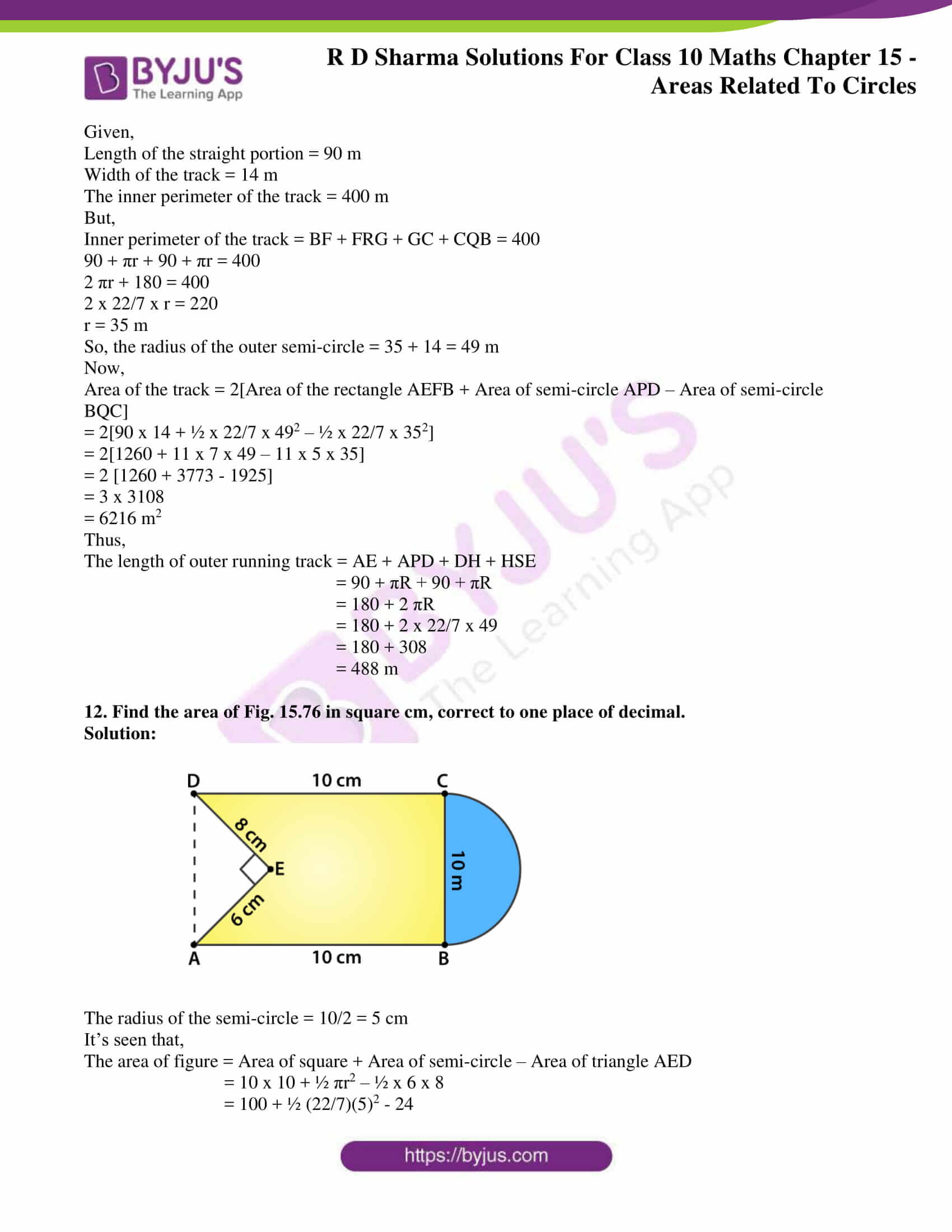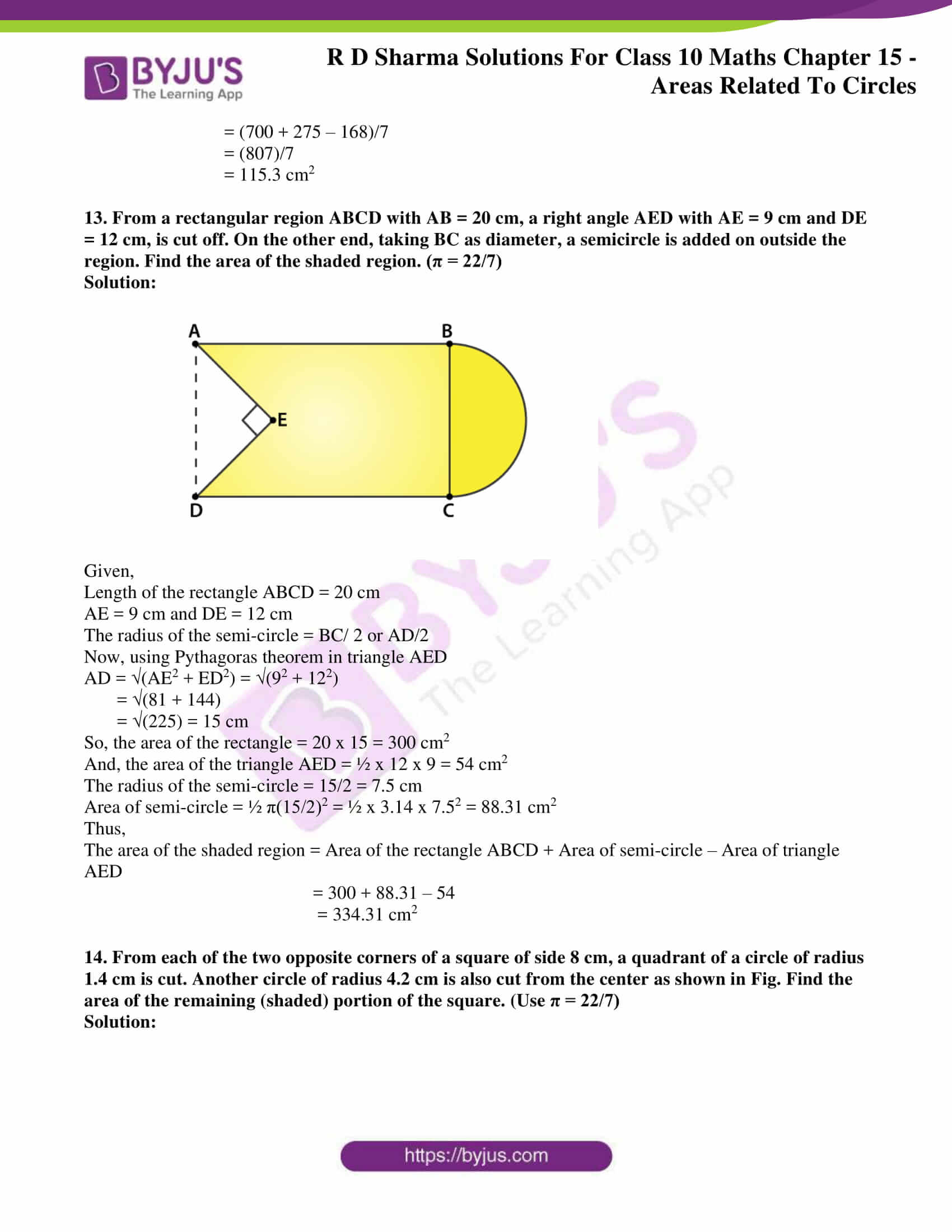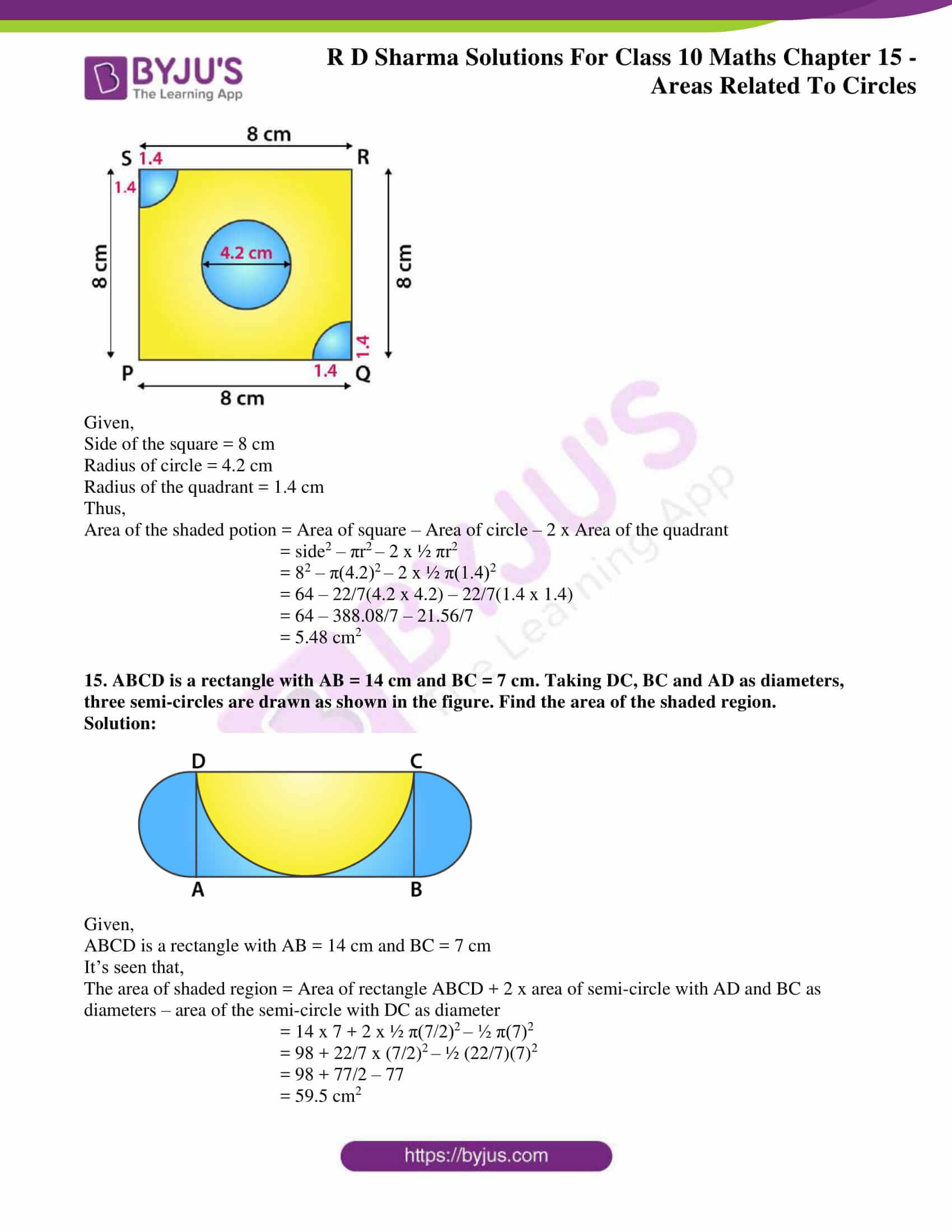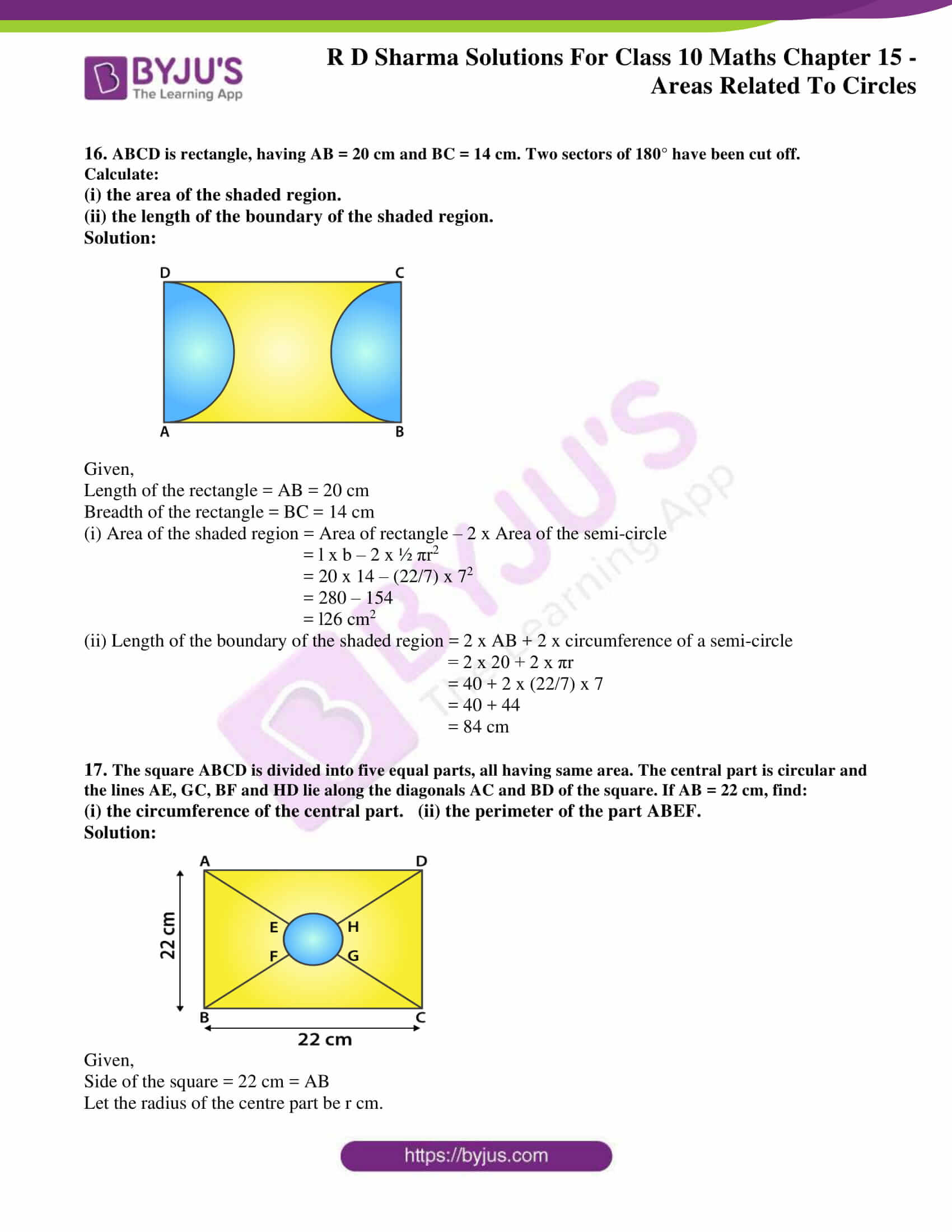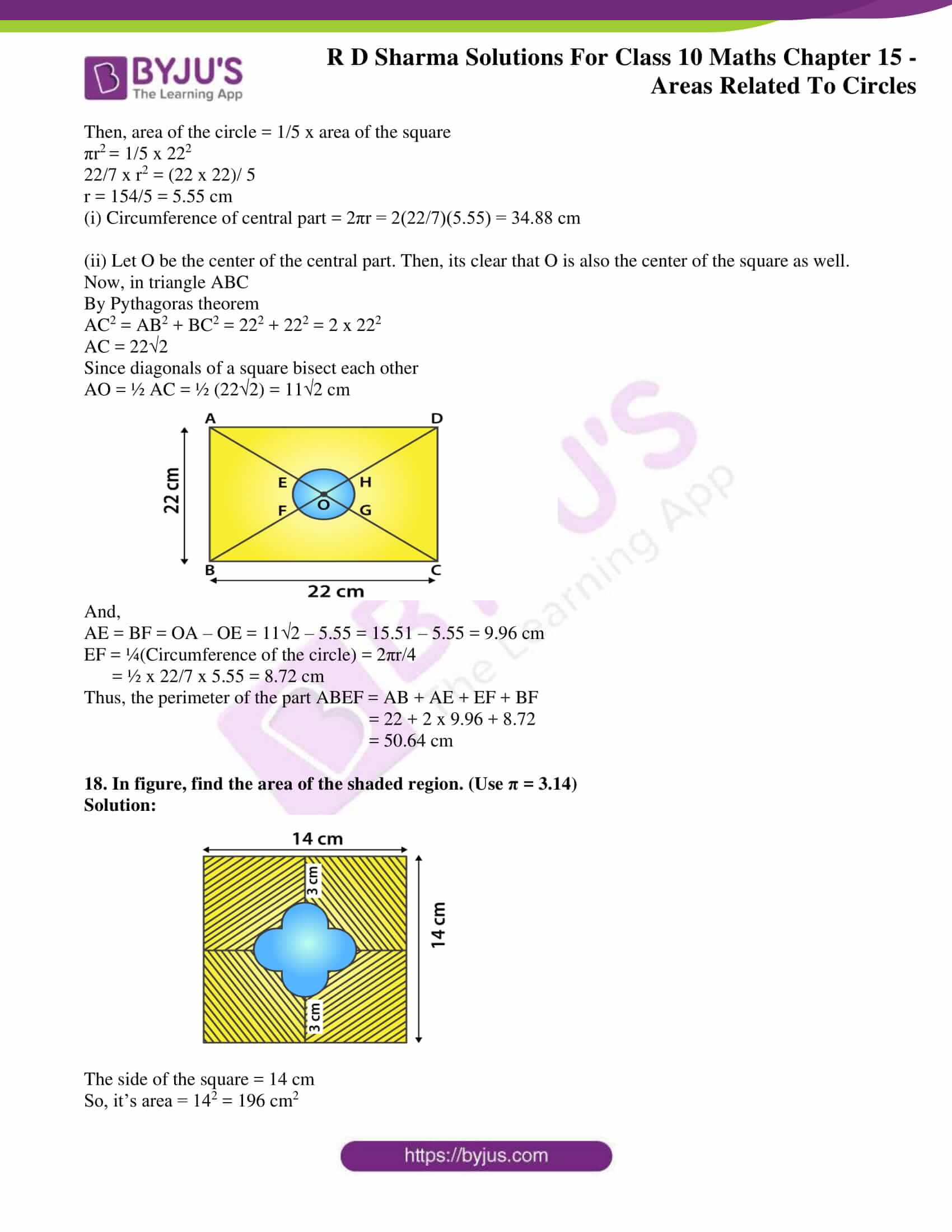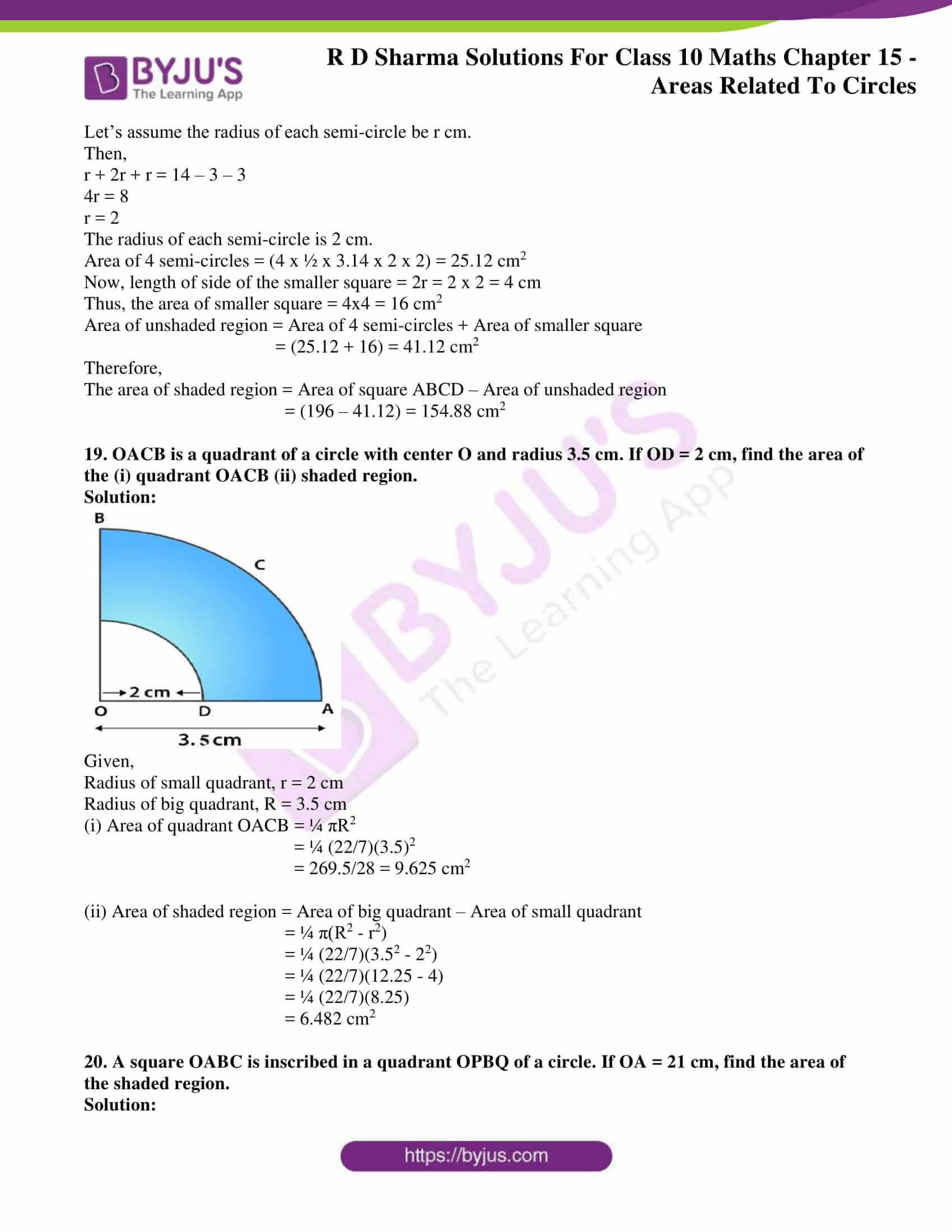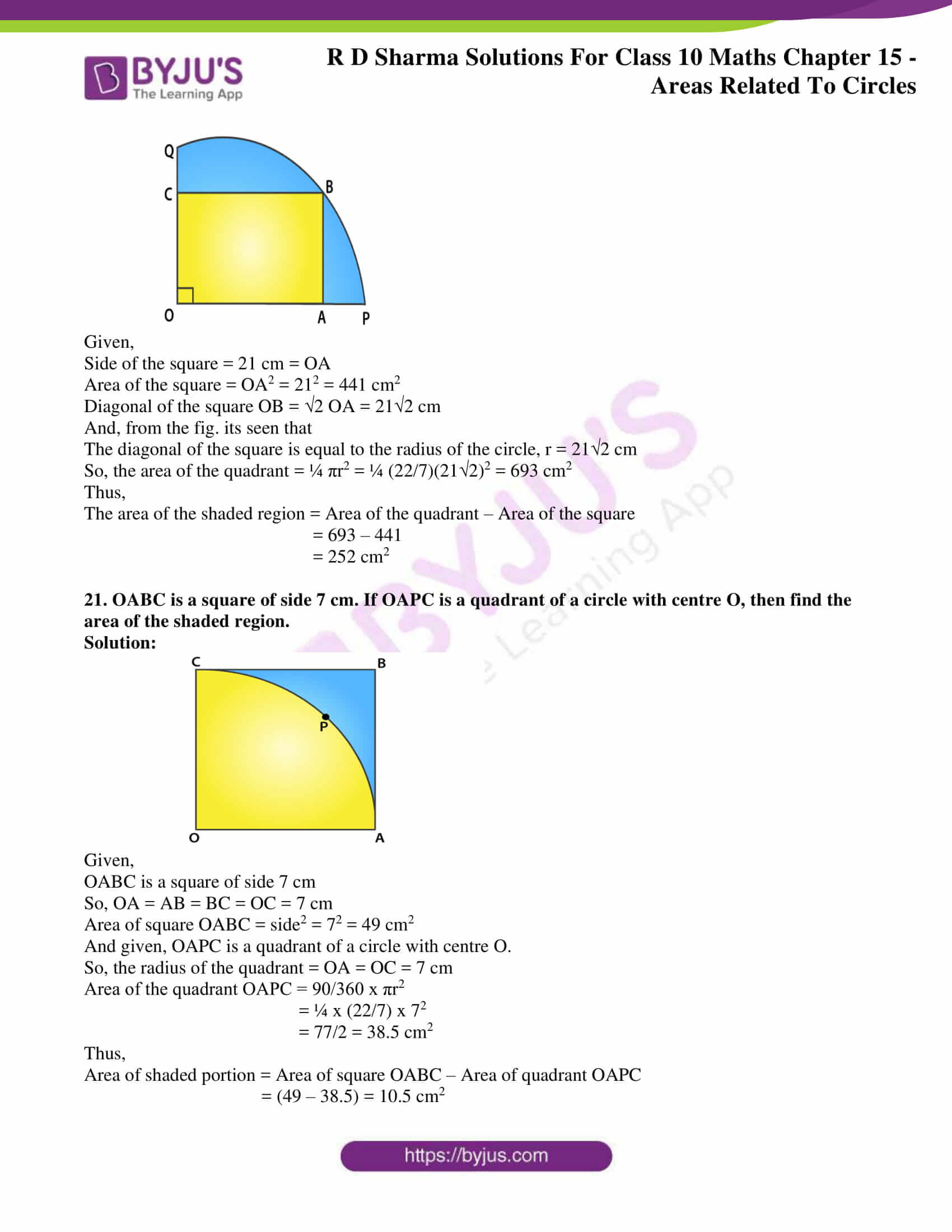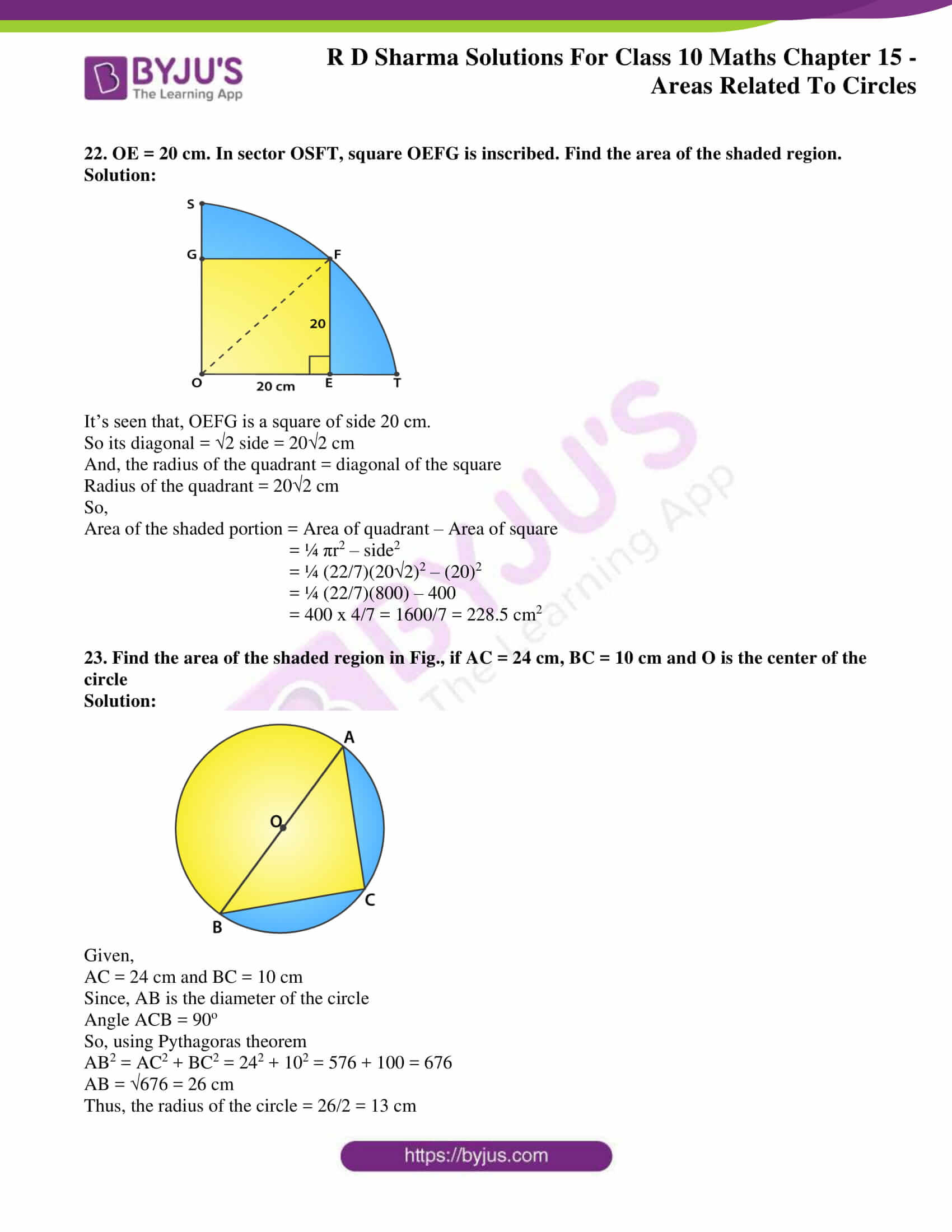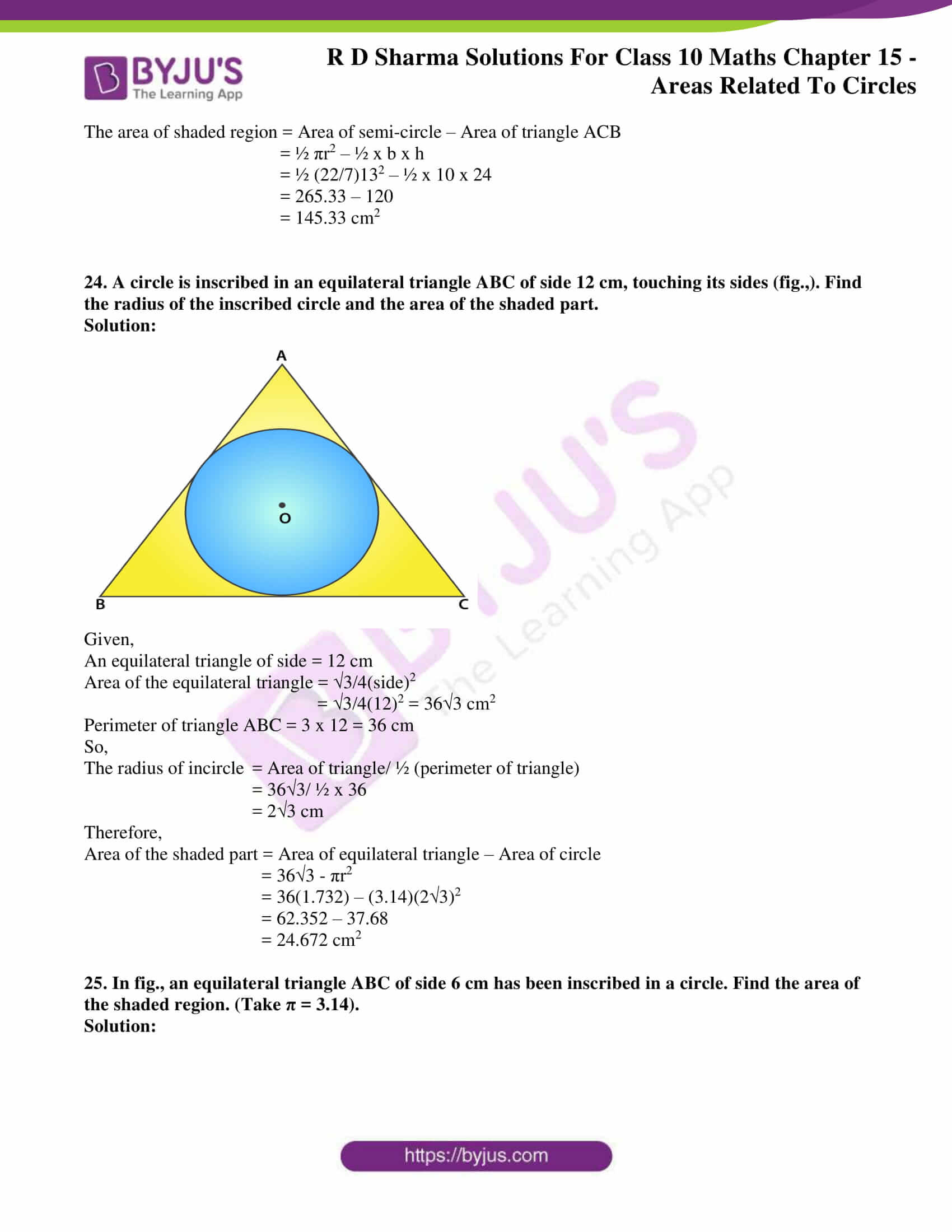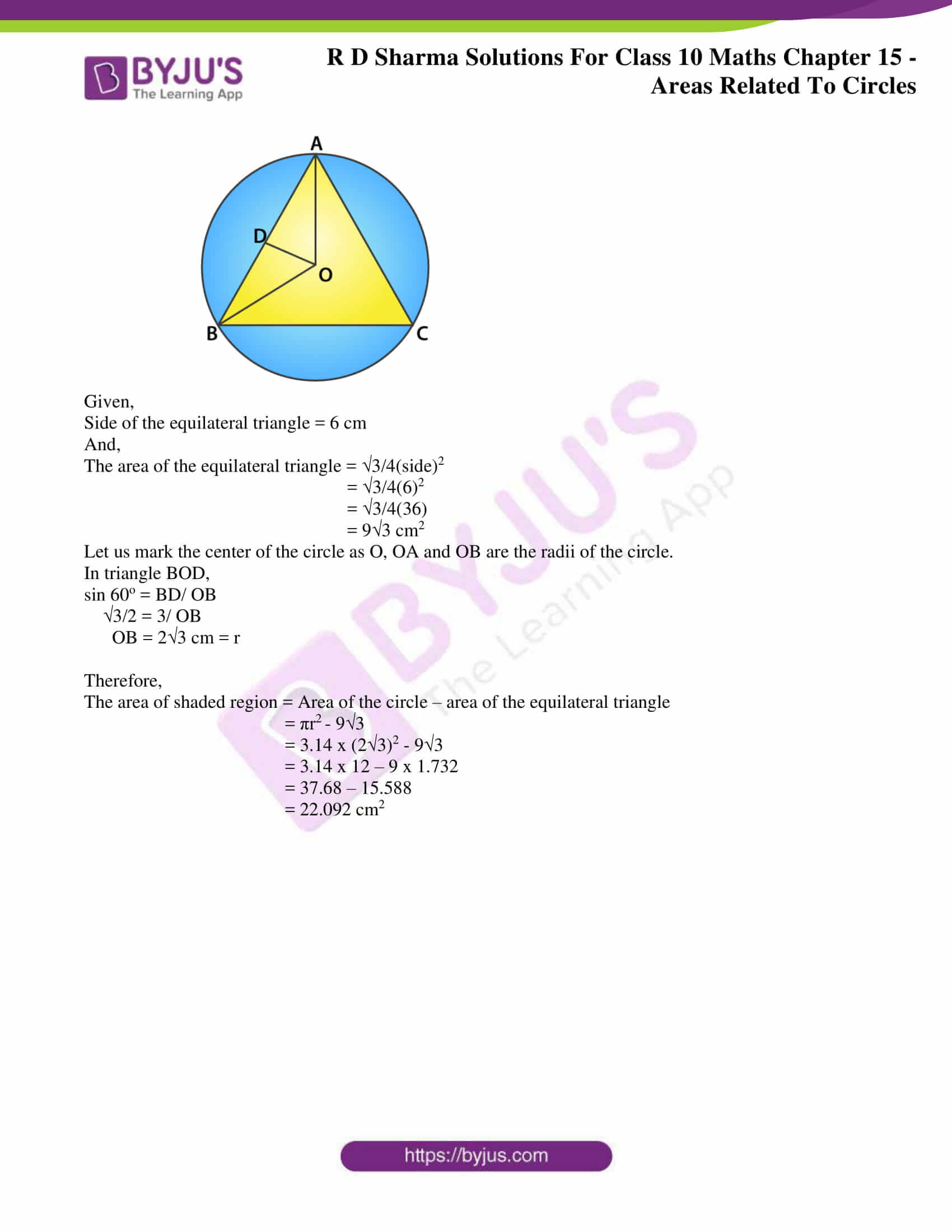### RD Sharma Solutions for Class 10 Maths Chapter 15 Exercise 15.1 Page No: 15.11

1. Find the circumference and area of a circle of radius of 4.2 cm.

Solution:

Given,

We know that,

Circumference of a circle = 2πr

= 2 × (22/7) × 4.2 = 26.4 cm2

Area of a circle = πr2

= (22/7) x 4.22

= 22 x 0.6 x 4.2 = 55.44 cm2

Hence, the circumference and area of the circle is 26.4 cm2 and 55.44 cm2.

2. Find the circumference of a circle whose area is 301.84 cm2

Solution:

Given,

Area of the circle = 301.84 cm2

We know that,

Area of a Circle = πr2 = 301.84 cm2

(22/7) × r2 = 301.84

r2 = 13.72 x 7 = 96.04

r = √96.04 = 9.8

So, the radius is = 9.8 cm.

Now, Circumference of a circle = 2πr

= 2 × (22/7) × 9.8 = 61.6 cm

Hence, the circumference of the circle is 61.6 cm.

3. Find the area of a circle whose circumference is 44 cm.

Solution:

Given,

Circumference = 44 cm

We know that,

Circumference of a circle = 2πr = 44 cm

2 × (22/7) × r = 44

r = 7 cm

Now, Area of a Circle = πr2

= (22/7) × 7 × 7

= 154 cm2

Hence, area of the Circle = 154 cm2

4. The circumference of a circle exceeds the diameter by 16.8 cm. Find the circumference of the circle.

Solution:

Let the radius of the circle be r cm

So, the diameter (d) = 2r [As radius is half the diameter]

We know that,

Circumference of a circle (C) = 2πr

From the question,

Circumference of the circle exceeds its diameter by 16.8 cm

C = d + 16.8

2πr = 2r + 16.8      [d = 2r]

2πr – 2r = 16.8

2r (π – 1) = 16.8

2r (3.14 – 1) = 16.8

r = 3.92 cm

Now, the circumference of the circle (C) = 2πr

C = 2 × 3.14 × 3.92

= 24.64 cm

Hence, the circumference of the circle is 24.64 cm.

5. A horse is tied to a pole with 28 m long string. Find the area where the horse can graze.

Solution:

Given,

Length of the string (l) = 28 m

Area the horse can graze is the area of the circle with a radius equal to the length of the string.

We know that,

Area of a Circle = πr2

= (22/7) × 28 × 28 = 2464 m2

Hence, the area of the circle which is same as the area the horse can graze is 2464 m2

6. A steel wire when bent in the form of a square encloses an area of 121 cm2. If the same wire is bent in the form of a circle, find the area of the circle.

Solution: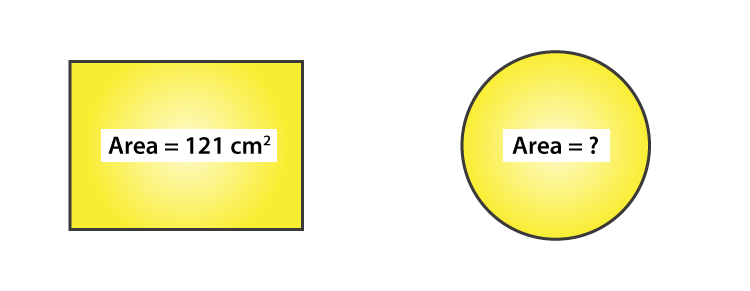Given,

Area of the square = a2 = 121 cm2

We know that,

Area of the circle = πr2

Area of a square = a2

121 cm2 = a2

So, a = 11 cm

Thus, each side of the square = 11 cm

Now, the perimeter of the square = 4a

= 4 × 11 = 44 cm

From the question, its understood that

Perimeter of the square = Circumference of the circle

We know that, circumference of a circle (C) = 2πr

4a = 2πr

44 = 2(22/7)r

r = 7 cm

Now, area of the Circle = πr2

= (22/7) × 7 × 7 = 154 cm2

Hence, the area of the circle is 154 cm2.

7. The circumference of two circles are in the ratio of 2:3. Find the ratio of their areas.

Solution:

Let’s consider the radius of two circles C1 and C2 be r1 and r2.

We know that, Circumference of a circle (C) = 2πr

And their circumference will be 2πr1 and 2πr2.

So, their ratio is = r1: r2

Given, circumference of two circles is in a ratio of 2: 3

r1: r2 = 2: 3

Then, the ratios of their areas is given as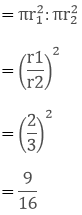= 4/9

Hence, ratio of their areas = 4: 9.

8. The sum of the radii of two circles is 140 cm and the difference of their circumference is 88 cm. Find the diameters of the circles.

Solution:

Let the radii of the two circles be r1 and r2.

And, the circumferences of the two circles be C1 and C2.

We know that, circumference of circle (C) = 2πr

Given,

Sum of radii of two circle i.e., r1 + r2 = 140 cm … (i)

Difference of their circumference,

C1 – C2 = 88 cm

2πr1 – 2πr= 88 cm

2(22/7)(r1 – r2) = 88 cm

(r1 – r2) = 14 cm

r1 = r2 + 14….. (ii)

Substituting the value of rin equation (i)we have,

r+ r2 + 14 = 140

2r2 = 140 – 14

2r= 126

r2 = 63 cm

Substituting the value of rin equation (ii)we have,

r= 63 + 14 = 77 cm

Therefore,

Diameter of circle 1 = 2r= 2 x 77 = 154 cm

Diameter of circle 2 = 2r= 2 × 63 = 126 cm

9. Find the radius of a circle whose circumference is equal to the sum of the circumferences of two circles of radii 15cm and 18cm.

Solution:

Given,

Radius of circle 1 = r1 = 15 cm

Radius of circle 2 = r2 = 18 cm

We know that, the circumference of a circle (C) = 2πr

So, C1 = 2πr1 and C2 = 2πr2

Let the radius be r of the circle which is to be found and its circumference (C)

Now, from the question

C = C1 + C2

2πr = 2πr1 + 2πr2

r = r1 + r2 [After dividing by 2π both sides]

r = 15 + 18

r = 33 cm

Thus, the radius of the circle = 33 cm

10. The radii of two circles are 8 cm and 6 cm respectively. Find the radius of the circle having its area equal to the sum of the areas of two circles.

Solution:

Given,

Radii of the two circles are 6 cm and 8 cm

Area of circle with radius 8 cm = π (8)2 = 64π cm2

Area of circle with radius 6cm = π (6)2 = 36π cm2

Sum of areas = 64π + 36π = 100π cm2

Let the radius of the circle be x cm

Area of the circle = 100π cm2 (from above)

πx= 100π

x = √100 = 10 cm

Therefore, the radius of the circle is 10 cm.

11. The radii of two circles are 19 cm and 9 cm respectively. Find the radius and area of the circle which has circumferences is equal to sum of the circumference of two circles.

Solution:

Given,

Radius of circle 1 = r1 = 19 cm

Radius of circle 2 = r2 = 9 cm

We know that, the circumference of a circle (C) = 2πr

So, C1 = 2πr1 and C2 = 2πr2

Let the radius be r of the circle which is to be found and its circumference (C)

Now, from the question

C = C1 + C2

2πr = 2πr1 + 2πr2

r = r1 + r2 [After dividing by 2π both sides]

r = 19 + 9

r = 28 cm

Thus, the radius of the circle = 28 cm

So, the area of required circle = πr2 = (22/7) × 28 × 28 = 2464 cm2

12. The area of a circular playground is 22176 m2. Find the cost of fencing this ground at the rate of ₹50 per metre.

Solution:

Given,

Area of the circular playground = 22176 m2

And the cost of fencing per metre = ₹50

If the radius of the ground is taken as r.

Then, its area = πr2

πr2 = 22176

r2 = 22176(7/22) = 7056

Taking square root on both sides, we have

r = 84 m

We know that, fencing is done only on the circumference of the ground

Circumference of the ground = 2πr = 2(22/7)84 = 528 m

So, the cost of fencing 528 m = ₹50 x 528 = ₹26400

Therefore, the cost of fencing the ground is ₹26400.

13. The side of a square is 10 cm. Find the area of the circumscribed and inscribed circles.

Solution:

For circumscribed circle:

Radius = diagonal of square/ 2

Diagonal of the square = side x √2

= 10√2 cm

Radius = (10 × 1.414)/ 2 = 7.07 cm

Thus, the radius of the circumcircle = 7.07 cm

Then, its area is = πr2 = (22/7) × 7.07 × 7.07 = 157.41 cm

Therefore, the Area of the Circumscribed circle is 157.41 cm

For inscribed circle:

Radius = side of square/ 2

= 10/ 2 = 5 m

Then, its area is = πr2 = 3.14 × 5 × 5 = 78.5 cm

Thus, the area of the circumscribed circle is 157.41 cmand the area of the inscribed circle is 78.5 cm2.

14. If a square is inscribed in a circle, find the ratio of areas of the circle and the square.

Solution: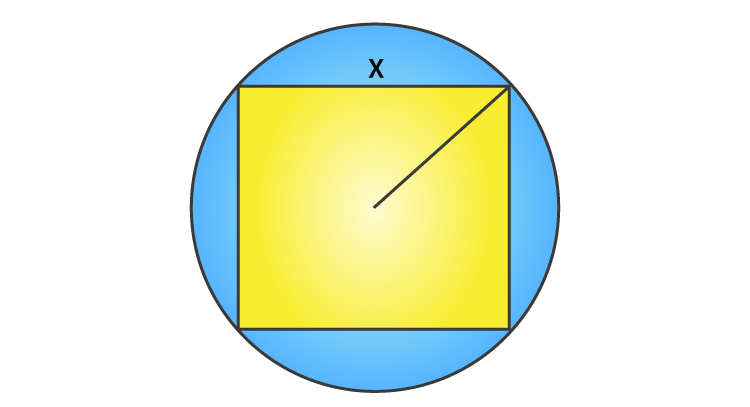Let side of square be x cm which is inscribed in a circle.

Given,

Radius of circle (r) = 1/2 (diagonal of square)

= 1/2(x√2)

r = x/√2

We know that, area of the square = x2

And, the area of the circle = πr2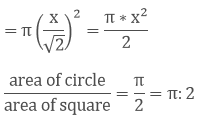Therefore, the ratio of areas of the circle and the square = π : 2

15. The area of circle inscribed in an equilateral triangle is 154 cm2. Find the perimeter of the triangle.

Solution: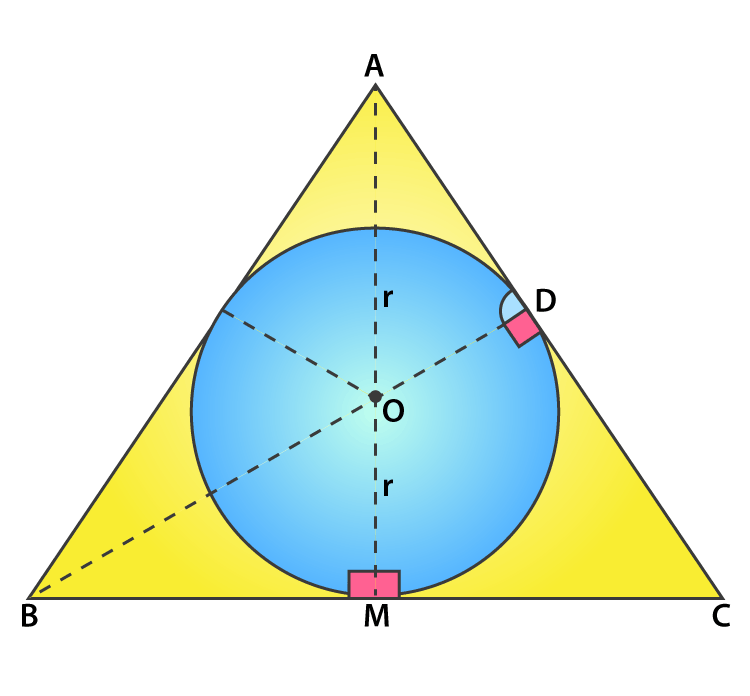Let the circle inscribed in the equilateral triangle be with a centre O and radius r.

We know that, Area of a Circle = πr2

But, the given that area is 154 cm2.

(22/7) × r2 = 154

r2 = (154 x 7)/22 = 7 × 7 = 49

r = 7 cm

From the figure seen above, we infer that

At point M, BC side is tangent and also at point M, BM is perpendicular to OM.

We know that,

In an equilateral triangle, the perpendicular from vertex divides the side into two halves.

BM = ½ x BC

Consider the side of the equilateral triangle be x cm.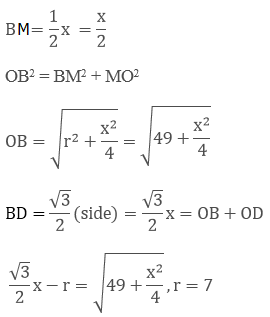After solving the above equation we get,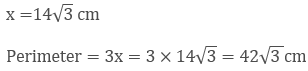Therefore, the perimeter of the triangle is found to be 42√3 cm = 42(1.73) = 72.7 cm

### RD Sharma Solutions for Class 10 Maths Chapter 15 Exercise 15.2 Page No: 15.24

1. Find, in terms of π, the length of the arc that subtends an angle of 30o at the centre of a circle of radius of 4 cm.

Solution:

Given,

Angle subtended at the centre ‘O’ = 30°

We know that,

Length of arc = θ/360 × 2πr cm

Length of arc = 30/360 × 2π∗4 cm = 2π/3 cm

Thus, the length of arc that subtends an angle of 30o degrees is 2π/3 cm

2. Find the angle subtended at the centre of a circle of radius 5 cm by an arc of length 5π/3 cm.

Solution:

Given,

Length of arc = 5π/3 cm

We know that,

Length of arc = θ/360 ∗ 2πr cm

5π/3 cm = θ/360 ∗ 2πr cm

Solving the above, we get

θ = 60°

Thus, the angle subtended at the centre of circle is 60°

3. An arc of length 20π cm subtends an angle of 144° at the centre of a circle. Find the radius of the circle.

Solution:

Given,

Length of arc = 20π cm

And. θ = Angle subtended at the centre of circle = 144°

We know that,

Length of arc = θ/360 ∗ 2πr cm

θ/360 ∗ 2πr cm = 144/360 ∗ 2πr cm = 4π/5 ∗ r cm

From the question, we can equate

20π cm = 4π/5 ∗ r cm

r = 25 cm.

Thus, the radius of the circle is 25 cm.

4. An arc of length 15 cm subtends an angle of 45° at the centre of a circle. Find in terms of π, the radius of the circle.

Solution:

Given,

Length of arc = 15 cm

θ = Angle subtended at the centre of circle = 45°

We know that,

Length of arc = θ/360 ∗ 2πr cm

= 45/360 ∗ 2πr cm

From the question, we can equate

15 cm = 45/360 ∗ 2π ∗ r cm

15 = πr/4

Radius = 15∗4/ π = 60/π

Therefore, the radius of the circle is 60/π cm.

5. Find the angle subtended at the centre of a circle of radius ‘a’ cm by an arc of length (aπ/4) cm.

Solution:

Given,

Length of arc = aπ/4 cm

θ = angle subtended at the centre of circle

We know that,

Length of arc = θ/360 ∗ 2πr cm

From the question, we can equate

θ/360 ∗ 2πa cm = aπ/4 cm

θ = 360/ (2 x 4)

θ = 45°

Hence, the angle subtended at the centre of circle is 45°

6. A sector of a circle of radius 4 cm subtends an angle of 30°. Find the area of the sector.

Solution:

Given,

Angle subtended at the centre O = 30°

We know that,

Area of the sector = θ/360 ∗ πr2

= 30/360 ∗ π42 =1/12 ∗ π16 = 4π/3

Therefore, the area of the sector of the circle = 4π/3 cm

7. A sector of a circle of radius 8 cm contains an angle of 135o. Find the area of sector.

Solution:

Given,

Angle subtended at the centre O = 135°

We know that,

Area of the sector = θ/360 ∗ πr2

Area of the sector = 135/360 ∗ π82

= 24π cm2

Therefore, Area of the sector calculated is 24π cm2

8. The area of a sector of a circle of radius 2 cm is π cm2. Find the angle contained by the sector.

Solution:

Given,

Angle subtended at the centre ‘O’

Area of sector of circle = π cm2

We know that,

Area of the sector = θ/360 ∗ πr2

= θ/360 ∗ π22

= πθ/90

From the question, we can equate

π  = π θ/90

On solving, we have

θ = 90°

Hence, the angle subtended at the centre of circle is 90°

9. The area of a sector of a circle of radius 5 cm is 5π cm2. Find the angle contained by the sector.

Solution:

Given,

Angle subtended at the centre ‘O’

Area of sector of circle = 5π cm2

We know that,

Area of the sector = θ/360 ∗ πr2

= θ/360 ∗ π52

= 5πθ/72

From the question, we can equate

= 5πθ/72

On solving, we have

θ = 72°

Hence, the angle subtended at the centre of circle is 72°

10. Find the area of the sector of a circle of radius 5 cm, if the corresponding arc length is 3.5 cm.

Solution:

Given,

Length of arc = 3.5 cm

Let θ = angle subtended at the centre of circle

We know that,

Length of arc = θ/360 ∗ 2πr cm

= θ/360 ∗ 2π(5)

From the question, we can equate

3.5 = θ/360 ∗ 2π(5)

3.5 = 10π ∗ θ/360

θ = 360 x 3.5/ (10π)

θ = 126/ π

Now, the area of the sector = θ/360 ∗ πr2

= (126/ π)/ 360 ∗ π(5)2

= 126 x 25 / 360 = 8.75

Hence, the area of the sector = 8.75 cm2

11. In a circle of radius 35 cm, an arc subtends an angle of 72° at the centre. Find the length of the arc and area of the sector.

Solution:

Given,

Angle subtended at the centre = 72°

We know that,

Length of arc = θ/360 ∗ 2πr cm

= 72/360 ∗ 2π(35) = 14π = 14(22/7) = 44 cm

Next,

Area of the sector = θ/360 ∗ πr2

= 72/360 ∗ π 352

= (0.2) x (22/7) x 35 x 35

= 0.2 x 22 x 5 x 35

Area of the sector = (35 × 22) = 770 cm2

Hence, the length of arc = 44cm and the area of the sector is 770 cm2.

12. The perimeter of a sector of a circle of radius 5.7 m is 27.2 m. Find the area of the sector.

Solution: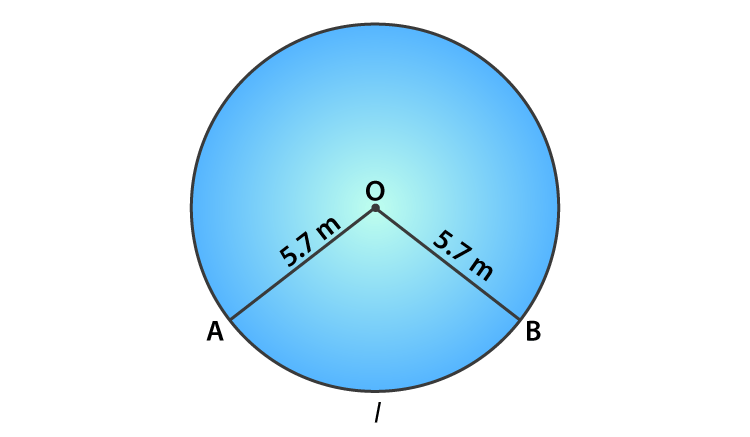Given,

Radius = 5.7 cm = OA = OB [from the figure shown above]

Perimeter of the sector = 27.2 m

Let the angle subtended at the centre be θ

We know that,

Length of arc = θ/360 ∗ 2πr m

Now, Perimeter of the sector = θ/360 ∗ 2πr + OA + OB

27.2 = θ/360 ∗ 2π x 5.7 cm + 5.7 + 5.7

27.2 – 11.4 = θ/360 ∗ 2π x 5.7

15.8 = θ/360 ∗ 2π x 5.7

θ = 158.8°

So, the area of the sector = θ/360 ∗ πr2

Area of the sector = 158.8/360 ∗ π 5.72

Solving the above, we get

Area of the sector = 45.03 m2

13. The perimeter of a certain sector of a circle of radius is 5.6 m and 27.2 m. Find the area of the sector.

Solution: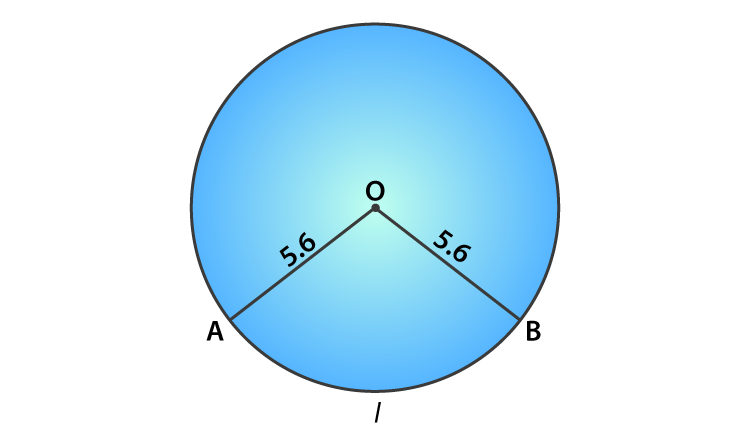Given,

Radius of the circle = 5.6 m = OA = OB

Perimeter of the sector = (AB arc length) + OA + OB = 27.2

Let the angle subtended at the centre be θ

We know that,

Length of arc = θ/360 ∗ 2πr cm

θ/360 ∗ 2πr cm + OA + OB = 27.2 m

θ/360 ∗ 2πr cm + 5.6 + 5.6 = 27.2 m

Solving the above, we get

θ = 163.64°

Now, the Area of the sector = θ/360 ∗ πr2

Area of the sector = 163.64/360 ∗ π 5.62 = 44.8

Therefore, the area of the sector = 44.8 m2

### RD Sharma Solutions for Class 10 Maths Chapter 15 Exercise 15.3 Page No: 15.32

1. AB is a chord of a circle with centre O and radius 4 cm. AB is of length 4 cm and divides the circle into two segments. Find the area of the minor segment.

Solution: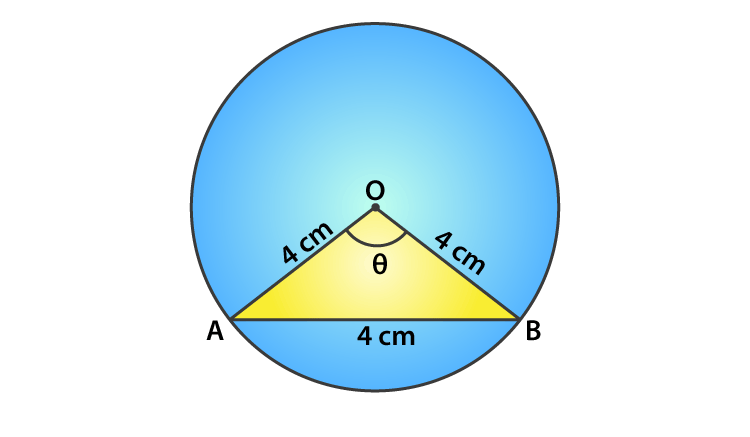Given,

Radius of the circle with centre ‘O’, r = 4 cm = OA = OB

Length of the chord AB = 4 cm

So, OAB is an equilateral triangle and angle AOB = 60°

Thus, the angle subtended at centre θ = 60°

Area of the minor segment = (Area of sector) – (Area of triangle AOB)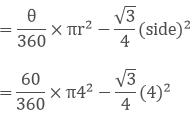= (8π/3 – 4√3) = 8.37 – 6.92 = 1.45 cm2

Therefore, the required area of the segment is (8π/3 – 4√3) cm2

2. A chord PQ of length 12 cm subtends an angle 120o at the centre of a circle. Find the area of the minor segment cut off by the chord PQ.

Solution: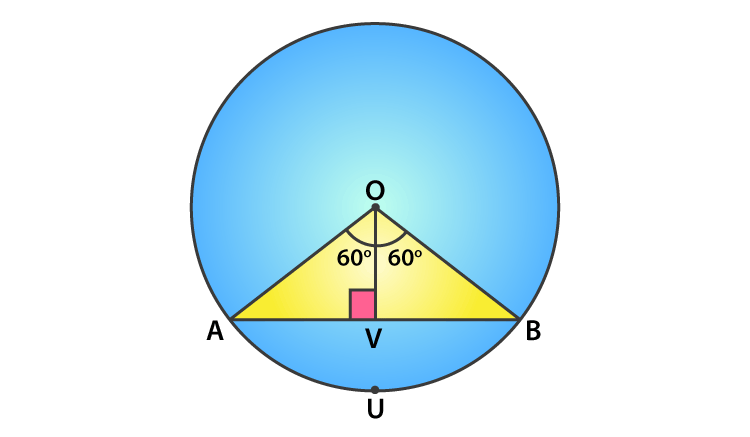Given, ∠POQ = 120o and PQ = 12 cm

Draw OV ⊥ PQ,

PV = PQ × (0.5) = 12 × 0.5 = 6 cm

Since, ∠POV = 120o

∠POV = ∠QOV = 60o

In triangle OPQ, we have

sin θ = PV/ OA

sin 60o = 6/ OA

√3/2 = 6/ OA

OA = 12/ √3 = 4√3 = r

Now, using the above we shall find the area of the minor segment

We know that,

Area of the segment = area of sector OPUQO – area of △OPQ

= θ/360 x πr2 – ½ x PQ x OV

= 120/360 x π(4√3)2 – ½ x 12 x 2√3

= 16π – 12√3 = 4(4π – 3√3)

Therefore, the area of the minor segment = 4(4π – 3√3) cm2

3. A chord of a circle of radius 14 cm makes a right angle at the centre. Find the areas of the minor and major segments of the circle.

Solution: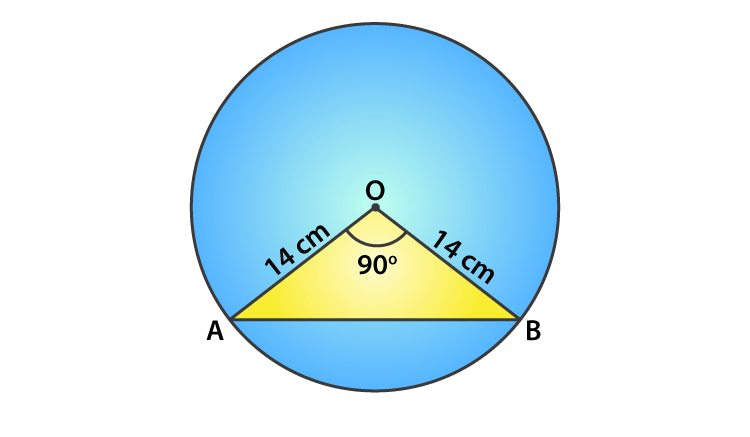Given,

Angle subtended by the chord with the centre of the circle, θ = 90°

Area of minor segment = θ/360 x πr2 – ½ x r2 sin θ

= 90/360 x π(14)2 – ½ x 142 sin (90)

= ¼ x (22/7) (14)2 – 7 x 14

= 56 cm2

Area of circle = πr2

= 22/7 x (14)2 = 616 cm2

Thus,

The area of the major segment = Area of circle – Area of the minor segment

= 616 – 56

= 560 cm2

4. A chord 10 cm long is drawn in a circle whose radius is 5√2 cm. Find the area of both the segments.

Solution: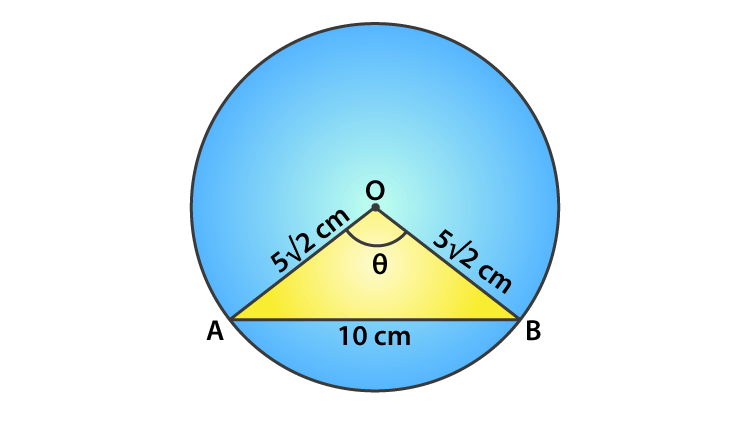Given,

Radius of the circle, r = 5√2 cm = OA = OB

Length of the chord AB = 10 cm

In triangle OAB,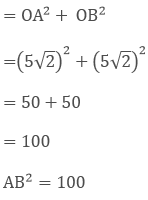We see that the Pythagoras theorem is satisfied.

So, OAB is a right angle triangle.

Angle subtended by the chord with the centre of the circle, θ = 90°

Area of minor segment = area of sector – area of triangle

= θ/360 × πr2 – ½ x r2 sin θ

= 90/360 × (3.14) 5√22 – ½ x (5√2)2 sin 90

= [¼ x 3.14 x 25 x 2] – [½ x 25 x 2 x 1]

= 25(1.57 – 1)

= 14.25 cm2

Area of circle = πr2 = 3.14 x (5√2)2 = 3.14 x 50 = 157 cm2

Thus, Area of major segment = Area of circle – Area of minor segment

= 157 – 14.25

= 142.75 cm2

5. A chord AB of circle of radius 14 cm makes an angle of 60° at the centre of a circle. Find the area of the minor segment of the circle.

Solution:

Given,

Radius of the circle (r) = 14 cm = OA = OB

Angle subtended by the chord with the centre of the circle, θ = 60°

In triangle AOB, angle A = angle B [angle opposite to equal sides OA and OB = x]

By angle sum property,

∠A + ∠B + ∠O = 180

x + x + 60° = 180°

2x = 120°, x = 60°

All angles are 60° so the triangle OAB is an equilateral with OA = OB = AB

Area of the minor segment = area of sector – area of triangle OAB

= θ/360 × πr2 – 1/2 r2 sin θ

= θ/360 × πr2 – 1/2 x (14)2 sin 60°

= 60/360 × (22/7) 142 – 1/2 x (14)2 x √3/2

= 60/360 x (22/7) 142 – √3/4 x (14)2

= 142 [(1/6) x (22/7)] – 0.4330

= 142 [(22/42)] – 0.4330

= 142 [(11-9.093)/21]

= 142 [0.09080]

= 17.79

Therefore, the area of the minor segment = 17.79 cm2

### RD Sharma Solutions for Class 10 Maths Chapter 15 Exercise 15.4 Page No: 15.56

1. A plot is in the form of a rectangle ABCD having semi-circle on BC as shown in Fig.15.64. If AB = 60m and BC = 28m, find the area of the plot.

Solution: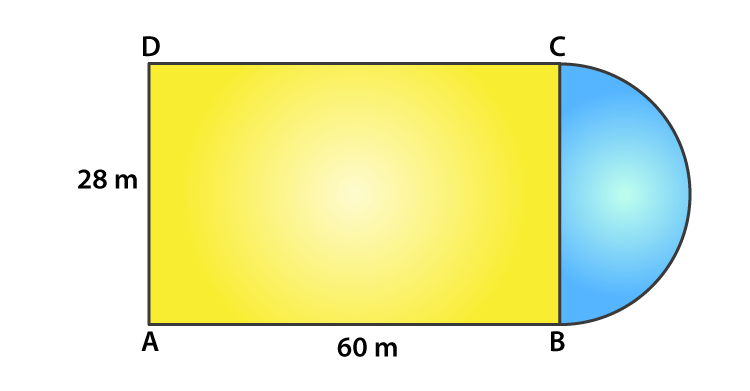Given, ABCD is a rectangle

So, AB = CD = 60 m

And, BC = AD = 28 m

For the radius of the semi-circle = BC/2 = 28/2 = 14 m

Now,

Area of the plot = Area of rectangle ABCD + Area of semi – circle

= (l x b) + ½ πr2

= (60 x 28) + ½ (22/7)(14)2

= 1680 + 308

= 1988 cm2

2. A play ground has the shape of a rectangle, with two semi-circles on its smaller sides as diameters, added to its outside. If the sides of the rectangle are 36m and 24.5m, find the area of the playground.

Solution: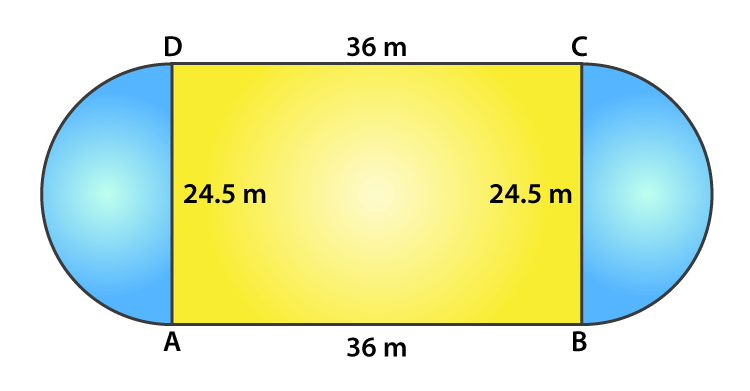Given,

Length of rectangle = 36 m

Breadth of rectangle = 24.5 m

Radius of the semi-circle = breath/2 = 24.5/2 = 12.25 m

Now,

Area of the playground = Area of the rectangle + 2 x area of semi-circles

= l x b + 2 x ½ (πr2)

= (36 x 24.5) + (22/7) x 12.252

= 882 + 471.625 = 1353.625

Thus, the area of the playground is 1353.625 m2

3. Find the area of the circle in which a square of area 64 cm2 is inscribed.

Solution: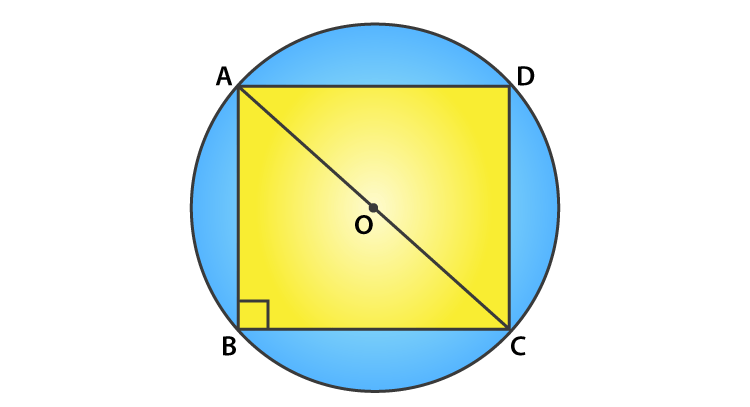Given,

Area of square inscribed the circle = 64 cm2

Side2 = 64

Side = 8 cm

So, AB = BC = CD = DA = 8 cm

In triangle ABC, by Pythagoras theorem we have

AC2 = AB2 + BC2

AC2 = 82 + 82

AC2 = 64 + 64 = 128

AC = √128 = 8√2 cm

Now, as angle B = 90o and AC being the diameter of the circle

The radius is AC/2 = 8√2/2 = 4√2 cm

Thus, the area of the circle = πr2 = 3.14(4√2)2

= 100.48 cm2

4. A rectangular piece is 20m long and 15m wide. From its four corners, quadrants of radii 3.5m have been cut. Find the area of the remaining part.

Solution: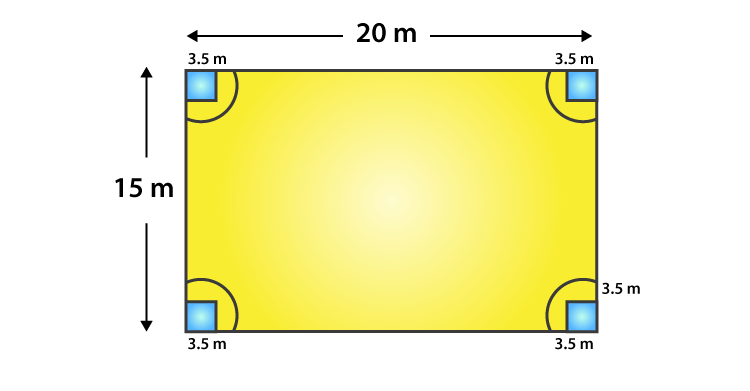Given,

Length of the rectangle = 20 m

Breadth of the rectangle = 15 m

So,

Area of the remaining part = Area of the rectangle – 4 x Area of one quadrant

= (l x b) – 4 x (¼ x πr2)

= (l x b) – πr2

= (20 x 15) – (22/7)(3.5)2

= 300 – 38.5

= 261.5 m2

5. In fig. 15.73, PQRS is a square of side 4 cm. Find the area of the shaded square.

Solution: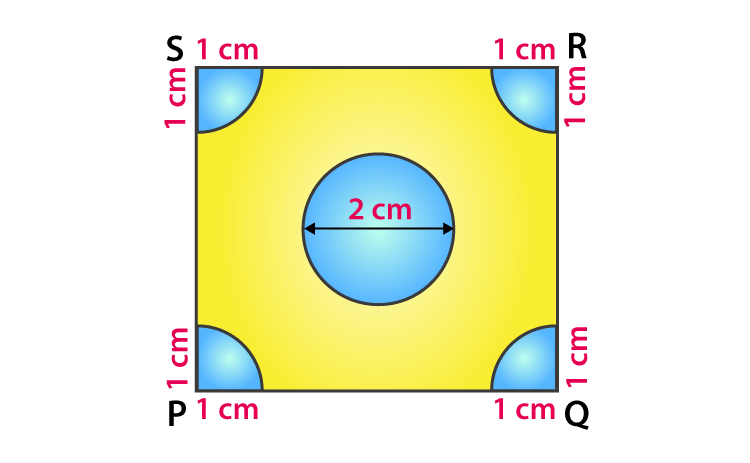We know that, each quadrant is a sector of 90o in a circle of 1 cm radius. In other words its 1/4th of a circle.

So, its area = ¼ x πr2

= ¼ x (22/7)(1)2 = 22/28 cm2

And, the area of the square = side2 [Given, side = 4 cm]

= 42 = 16 cm2

Area of the circle = πr2 = π(1)2 = 22/7 cm2  [Given, diameter = 2 cm, so radius = 1cm]

Thus,

The area of the shaded region = area of the square – area of the circle – 4 x area of a quadrant

= 16 – 22/7 – (4 x 22/28)

= 16 – 22/7 – 22/7 = 16 – 44/7

= 68/7 cm2

6. Four cows are tethered at four corners of a square plot of side 50m, so that they just cannot reach one another. What area will be left un-grazed?

Solution: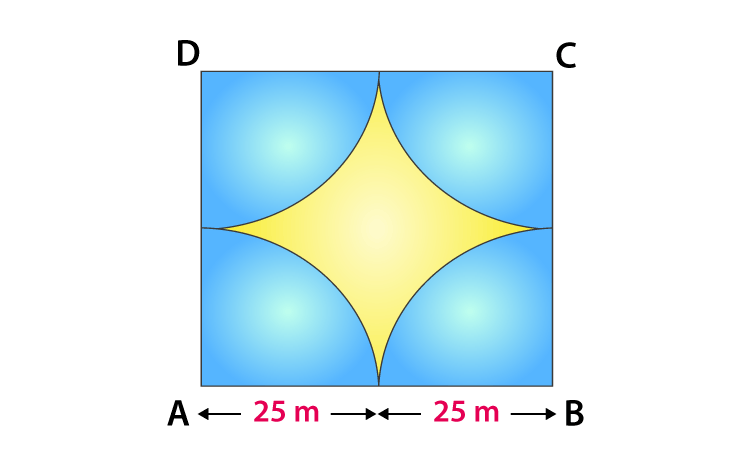Given,

Side of square plot = 50 m

So, we can tell

Area of plot left un-grazed = Area of the plot – 4 x (area of a quadrant)

= Side2 – 4 x (¼ x πr2)

= 502 – 22/7 x (25)2

= 2500 – 1964.28

= 535.72 m2

7. A cow is tied with a rope of length 14 m at the corner of a rectangle field of dimensions 20 m x 16 m, find the area of the field in which the cow can graze.

Solution: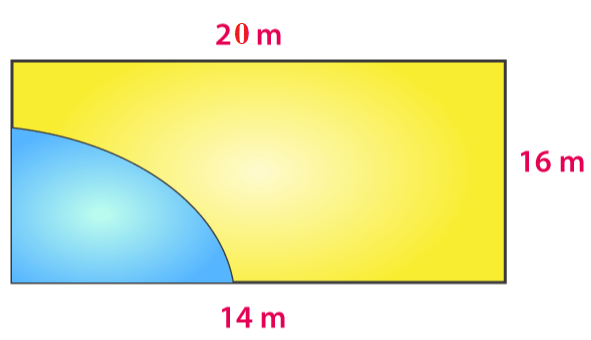The dotted portion indicated the area over which the cow can graze.

It’s clearly seen that, the shaded area is the area of a quadrant of a circle of radius equal to the length of the rope.

Thus, the required area = ¼ x πr2

= ¼ x 22/7 x 14 x 14

= 154

Hence, the area of the field in which the cow can graze is 154 cm2

8. A calf is tied with a rope of length 6 m at the corner of a square grassy lawn of side 20 m. If the length of the rope is increased by 5.5 m, find the increase in area of the grassy lawn in which the calf can graze.

Solution: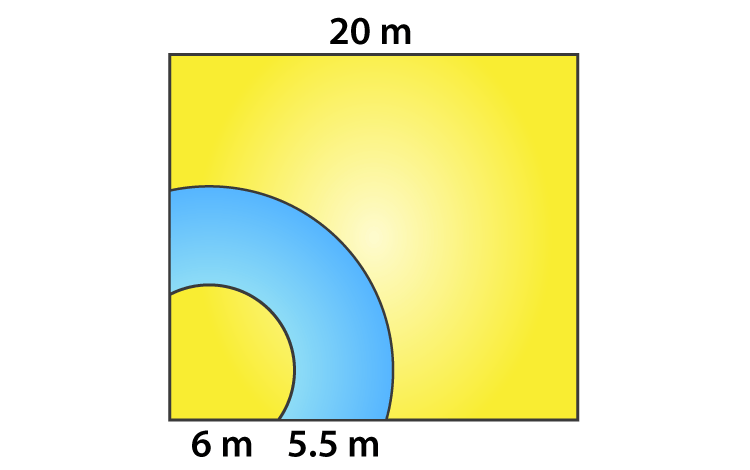Given,

The initial length of the rope = 6 m

Then the rope is said to be increased by 5.5m

So, the increased length of the rope = (6 + 5.5) = 11.5 m

We know that, the corner of the lawn is a quadrant of a circle.

Thus,

The required area = ¼ x π(11.5)2 – ¼ x π(5.5)2

= ¼ x 22/7 (11.52 – 62)

= ¼ x 22/7 (132.25 – 36)

= ¼ x 22/7 x 96.25

= 75.625 cm2

9. A square tank has its side equal to 40 m. There are four semi-circular grassy plots all around it. Find the cost of turfing the plot at Rs 1.25 per square meter.

Solution: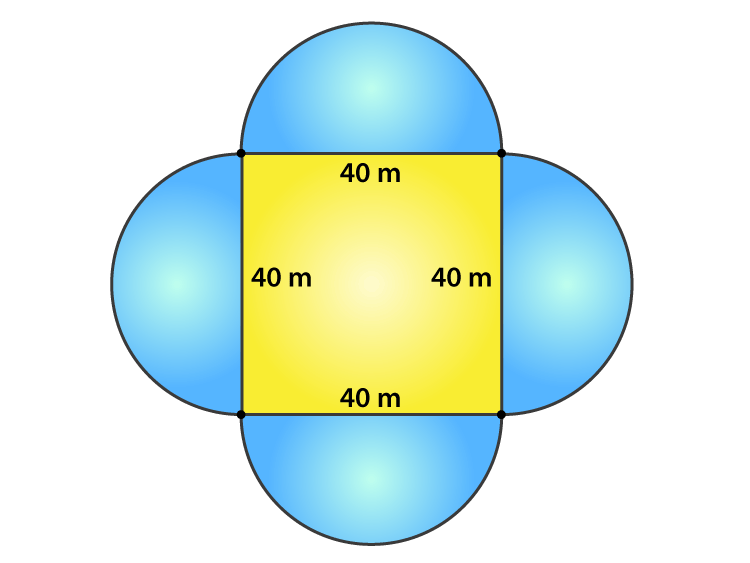Given,

Side of the square tank = 40 m

And, the diameter of the semi-circular grassy plot = side of the square tank = 40 m

Radius of the grassy plot = 40/2 = 20 m

Then,

The area of the four semi-circular grassy plots = 4 x ½ πr2

= 4 x ½ (3.14)(20)2

= 2512 m2

Rate of turfing the plot = Rs 1.25 per m2

So, the cost for 2512 m2 = (1.25 x 2512) = Rs 3140

10. A rectangular park is 100 m by 50 m. It is surrounded by semi-circular flower beds all round. Find the cost of levelling the semi-circular flower beds at 60 paise per square meter.

Solution: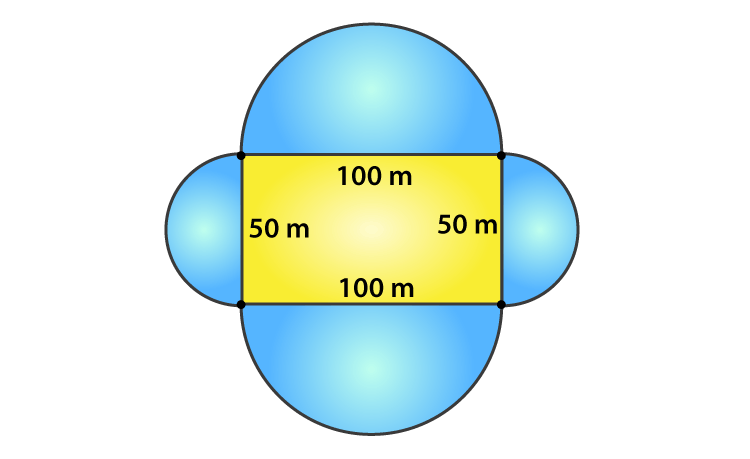Given,

Length of the park = 100 m and the breadth of the park = 50 m

The radius of the semi-circular flower beds = half of the corresponding side of the rectangular park

Radius of the bigger flower bed = 100/2 = 50 m

And the radius of the smaller flower bed = 50/2 = 25 m

Total area of the flower beds = 2[Area of bigger flower bed + Area of smaller flower bed]

= 2[½ π(50)2 + ½ π(25)2]

= π[(50)2 + (25)2]

= 3.14 x [2500 + 625]

= 9812.5 m2

Now, rate of levelling flower bed = 60 paise per m2

Therefore,

The total cost of levelling = 9812.5 x 60 = 588750 paise

= Rs 5887.50

11. The inner perimeter of a running track (show in Fig.) is 400 m. The length of each of the straight portion is 90 m and the ends are semi-circles. If the track is everywhere 14 m wide, find the area of the track. Also, find the length of the outer running track.

Solution: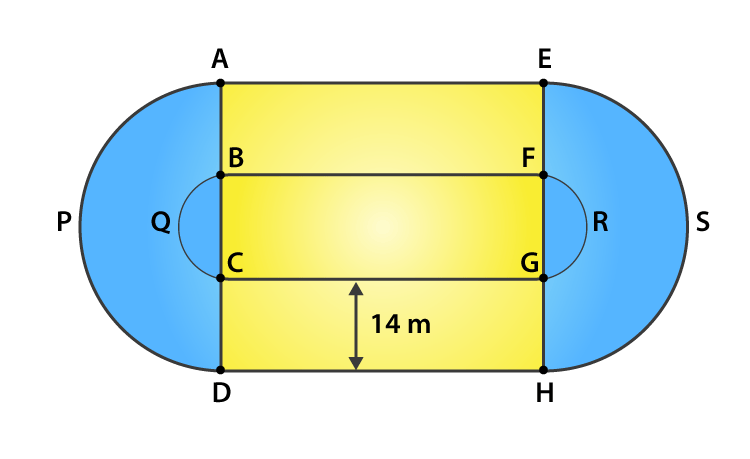Let the radius of the inner semi-circle = r

And that of the outer semi-circle = R

Given,

Length of the straight portion = 90 m

Width of the track = 14 m

The inner perimeter of the track = 400 m

But,

Inner perimeter of the track = BF + FRG + GC + CQB = 400

90 + πr + 90 + πr = 400

2 πr + 180 = 400

2 x 22/7 x r = 220

r = 35 m

So, the radius of the outer semi-circle = 35 + 14 = 49 m

Now,

Area of the track = 2[Area of the rectangle AEFB + Area of semi-circle APD – Area of semi-circle BQC]

= 2[90 x 14 + ½ x 22/7 x 492 – ½ x 22/7 x 352]

= 2[1260 + 11 x 7 x 49 – 11 x 5 x 35]

= 2 [1260 + 3773 – 1925]

= 3 x 3108

= 6216 m2

Thus,

The length of outer running track = AE + APD + DH + HSE

= 90 + πR + 90 + πR

= 180 + 2 πR

= 180 + 2 x 22/7 x 49

= 180 + 308

= 488 m

12. Find the area of Fig. 15.76 in square cm, correct to one place of decimal.

Solution: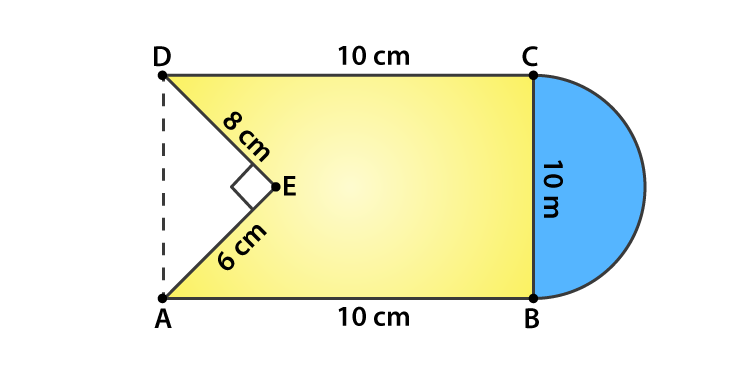The radius of the semi-circle = 10/2 = 5 cm

It’s seen that,

The area of figure = Area of square + Area of semi-circle – Area of triangle AED

= 10 x 10 + ½ πr2 – ½ x 6 x 8

= 100 + ½ (22/7)(5)2 – 24

= (700 + 275 – 168)/7

= (807)/7

= 115.3 cm2

13. From a rectangular region ABCD with AB = 20 cm, a right angle AED with AE = 9 cm and DE = 12 cm, is cut off. On the other end, taking BC as diameter, a semicircle is added on outside the region. Find the area of the shaded region. (π = 22/7)

Solution: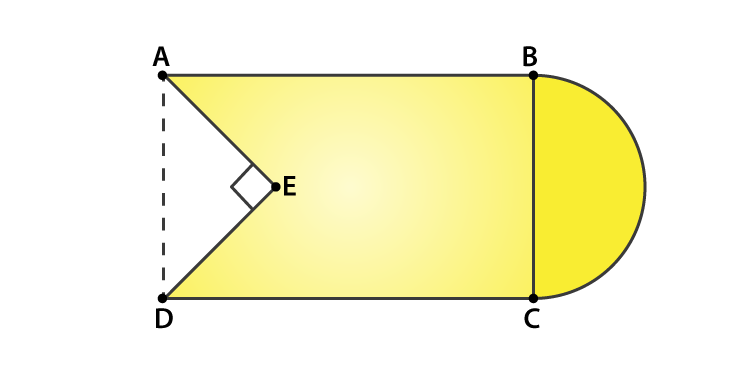Given,

Length of the rectangle ABCD = 20 cm

AE = 9 cm and DE = 12 cm

Now, using Pythagoras theorem in triangle AED

AD = √(AE2 + ED2) = √(92 + 122)

= √(81 + 144)

= √(225) = 15 cm

So, the area of the rectangle = 20 x 15 = 300 cm2

And, the area of the triangle AED = ½ x 12 x 9 = 54 cm2

The radius of the semi-circle = 15/2 = 7.5 cm

Area of semi-circle = ½ π(15/2)2 = ½ x 3.14 x 7.52 = 88.31 cm2

Thus,

The area of the shaded region = Area of the rectangle ABCD + Area of semi-circle – Area of triangle AED

= 300 + 88.31 – 54

= 334.31 cm2

14. From each of the two opposite corners of a square of side 8 cm, a quadrant of a circle of radius 1.4 cm is cut. Another circle of radius 4.2 cm is also cut from the center as shown in Fig. Find the area of the remaining (shaded) portion of the square. (Use π = 22/7)

Solution: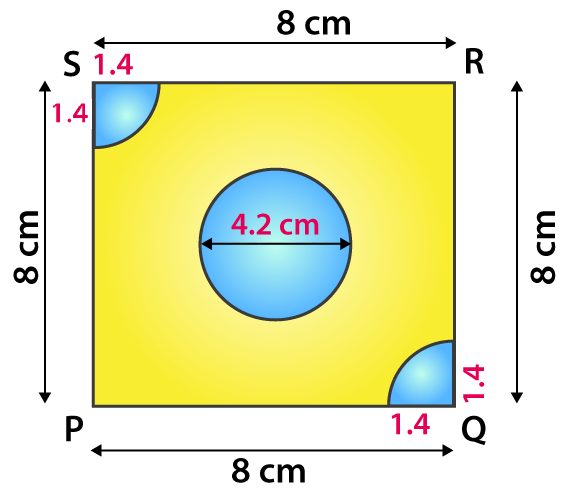Given,

Side of the square = 8 cm

Radius of circle = 4.2 cm

Thus,

Area of the shaded potion = Area of square – Area of circle – 2 x Area of the quadrant

= side2 – πr2 – 2 x ½ πr2

= 82 – π(4.2)2 – 2 x ½ π(1.4)2

= 64 – 22/7(4.2 x 4.2) – 22/7(1.4 x 1.4)

= 64 – 388.08/7 – 21.56/7

= 5.48 cm2

15. ABCD is a rectangle with AB = 14 cm and BC = 7 cm. Taking DC, BC and AD as diameters, three semi-circles are drawn as shown in the figure. Find the area of the shaded region.

Solution: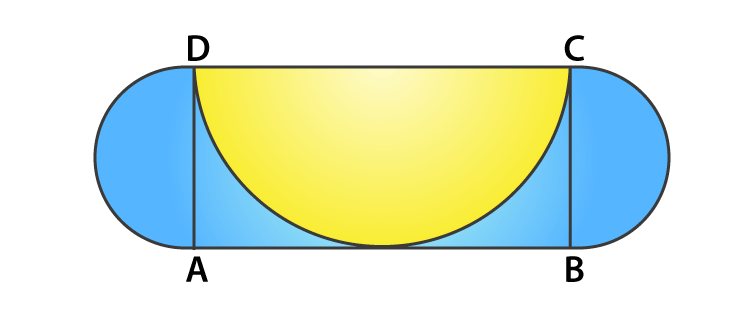Given,

ABCD is a rectangle with AB = 14 cm and BC = 7 cm

It’s seen that,

The area of shaded region = Area of rectangle ABCD + 2 x area of semi-circle with AD and BC as diameters – area of the semi-circle with DC as diameter

= 14 x 7 + 2 x ½ π(7/2)2 – ½ π(7)2

= 98 + 22/7 x (7/2)2 – ½ (22/7)(7)2

= 98 + 77/2 – 77

= 59.5 cm2

16. ABCD is rectangle, having AB = 20 cm and BC = 14 cm. Two sectors of 180° have been cut off. Calculate:

(i) the area of the shaded region.

(ii) the length of the boundary of the shaded region.

Solution: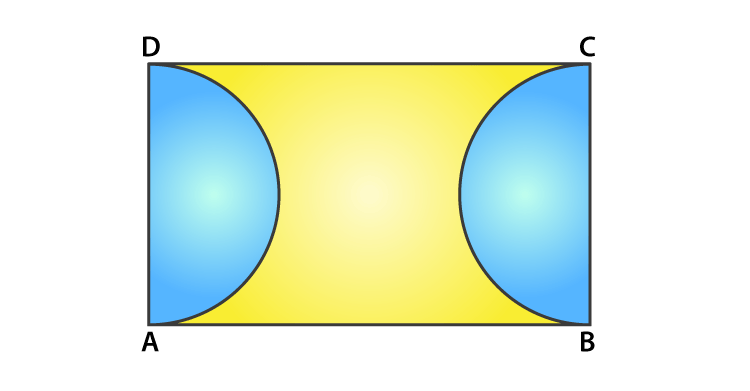Given,

Length of the rectangle = AB = 20 cm

Breadth of the rectangle = BC = 14 cm

(i) Area of the shaded region = Area of rectangle – 2 x Area of the semi-circle

= l x b – 2 x ½ πr2

= 20 x 14 – (22/7) x 72

= 280 – 154

= l26 cm2

(ii) Length of the boundary of the shaded region = 2 x AB + 2 x circumference of a semi-circle

= 2 x 20 + 2 x πr

= 40 + 2 x (22/7) x 7

= 40 + 44

= 84 cm

17. The square ABCD is divided into five equal parts, all having same area. The central part is circular and the lines AE, GC, BF and HD lie along the diagonals AC and BD of the square. If AB = 22 cm, find:

(i) the circumference of the central part.   (ii) the perimeter of the part ABEF.

Solution: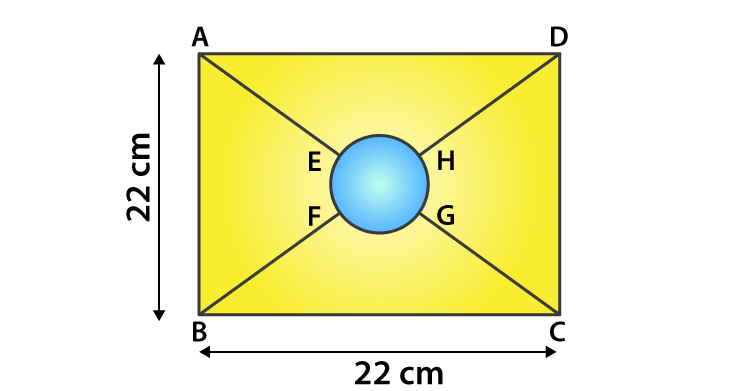Given,

Side of the square = 22 cm = AB

Let the radius of the centre part be r cm.

Then, area of the circle = 1/5 x area of the square

πr2 = 1/5 x 222

22/7 x r2 = (22 x 22)/ 5

r = 154/5 = 5.55 cm

(i) Circumference of central part = 2πr = 2(22/7)(5.55) = 34.88 cm

(ii) Let O be the center of the central part. Then, its clear that O is also the center of the square as well.

Now, in triangle ABC

By Pythagoras theorem

AC2 = AB2 + BC2 = 222 + 222 = 2 x 222

AC = 22√2

Since diagonals of a square bisect each other

AO = ½ AC = ½ (22√2) = 11√2 cm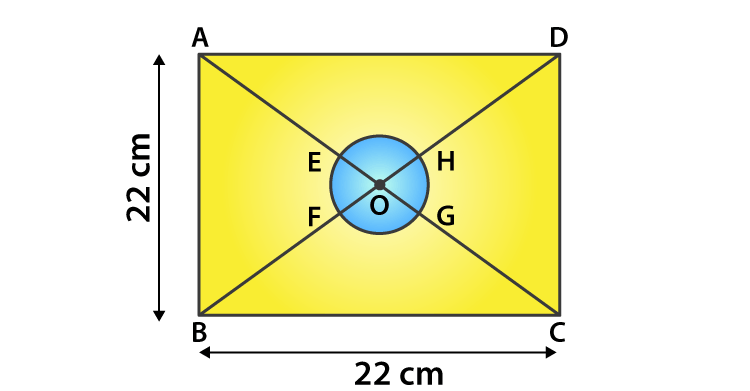And,

AE = BF = OA – OE = 11√2 – 5.55 = 15.51 – 5.55 = 9.96 cm

EF = ¼(Circumference of the circle) = 2πr/4

= ½ x 22/7 x 5.55 = 8.72 cm

Thus, the perimeter of the part ABEF = AB + AE + EF + BF

= 22 + 2 x 9.96 + 8.72

= 50.64 cm

18. In figure, find the area of the shaded region. (Use π = 3.14)

Solution: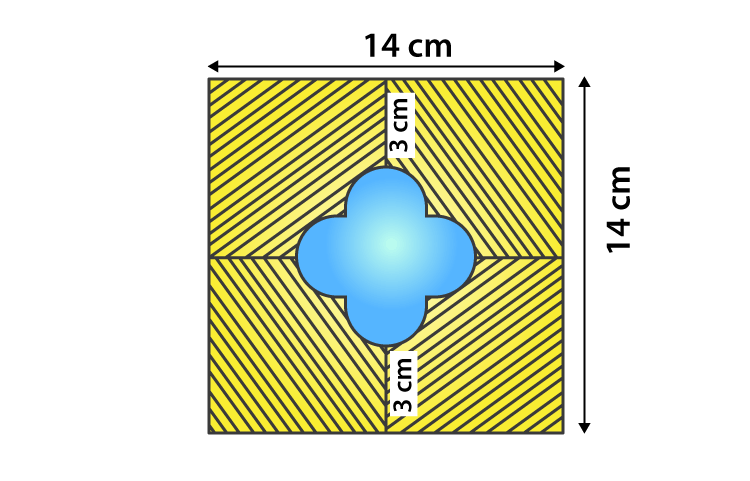The side of the square = 14 cm

So, it’s area = 142 = 196 cm2

Let’s assume the radius of each semi-circle be r cm.

Then,

r + 2r + r = 14 – 3 – 3

4r = 8

r = 2

The radius of each semi-circle is 2 cm.

Area of 4 semi-circles = (4 x ½ x 3.14 x 2 x 2) = 25.12 cm2

Now, length of side of the smaller square = 2r = 2 x 2 = 4 cm

Thus, the area of smaller square = 4×4 = 16 cm2

Area of unshaded region = Area of 4 semi-circles + Area of smaller square

= (25.12 + 16) = 41.12 cm2

Therefore,

The area of shaded region = Area of square ABCD – Area of unshaded region

= (196 – 41.12) = 154.88 cm2

19. OACB is a quadrant of a circle with center O and radius 3.5 cm. If OD = 2 cm, find the area of the (i) quadrant OACB (ii) shaded region.

Solution: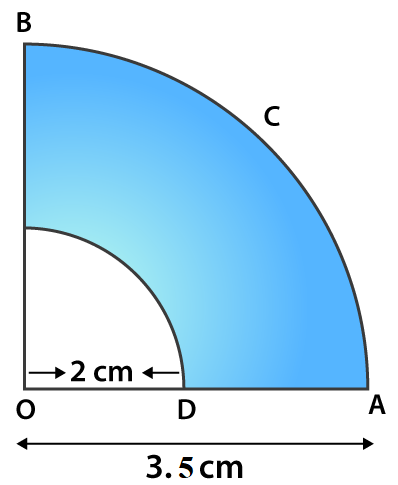Given,

(i) Area of quadrant OACB = ¼ πR2

= ¼ (22/7)(3.5)2

= 269.5/28 = 9.625 cm2

= ¼ π(R2 – r2)

= ¼ (22/7)(3.52 – 22)

= ¼ (22/7)(12.25 – 4)

= ¼ (22/7)(8.25)

= 6.482 cm2

20. A square OABC is inscribed in a quadrant OPBQ of a circle. If OA = 21 cm, find the area of the shaded region.

Solution:Given,

Side of the square = 21 cm = OA

Area of the square = OA2 = 212 = 441 cm2

Diagonal of the square OB = √2 OA = 21√2 cm

And, from the fig. its seen that

The diagonal of the square is equal to the radius of the circle, r = 21√2 cm

So, the area of the quadrant = ¼ πr2 = ¼ (22/7)(21√2)2 = 693 cm2

Thus,

The area of the shaded region = Area of the quadrant – Area of the square

= 693 – 441

= 252 cm2

21. OABC is a square of side 7 cm. If OAPC is a quadrant of a circle with centre O, then find the area of the shaded region.

Solution: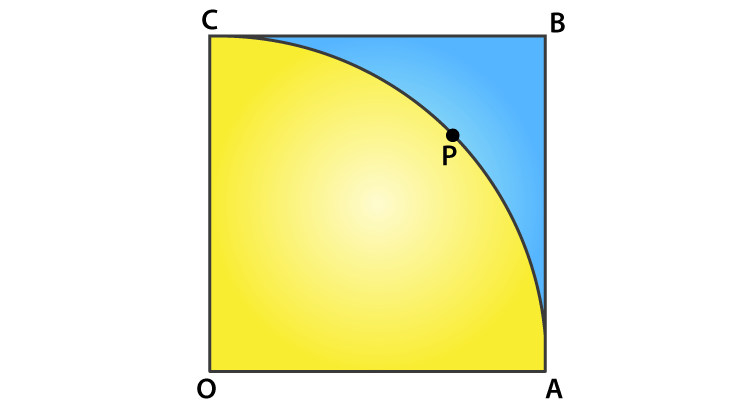Given,

OABC is a square of side 7 cm

So, OA = AB = BC = OC = 7 cm

Area of square OABC = side2 = 72 = 49 cm2

And given, OAPC is a quadrant of a circle with centre O.

So, the radius of the quadrant = OA = OC = 7 cm

Area of the quadrant OAPC = 90/360 x πr2

= ¼ x (22/7) x 72

= 77/2 = 38.5 cm2

Thus,

Area of shaded portion = Area of square OABC – Area of quadrant OAPC

= (49 – 38.5) = 10.5 cm2

22. OE = 20 cm. In sector OSFT, square OEFG is inscribed. Find the area of the shaded region.

Solution: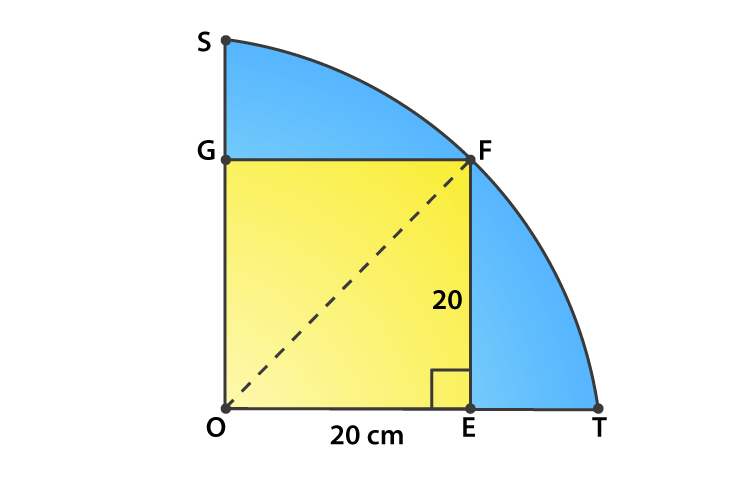It’s seen that, OEFG is a square of side 20 cm.

So its diagonal = √2 side = 20√2 cm

So,

Area of the shaded portion = Area of quadrant – Area of square

= ¼ πr2 – side2

= ¼ (22/7)(20√2)2 – (20)2

= ¼ (22/7)(800) – 400

= 400 x 4/7 = 1600/7 = 228.5 cm2

23. Find the area of the shaded region in Fig., if AC = 24 cm, BC = 10 cm and O is the center of the circle

Solution: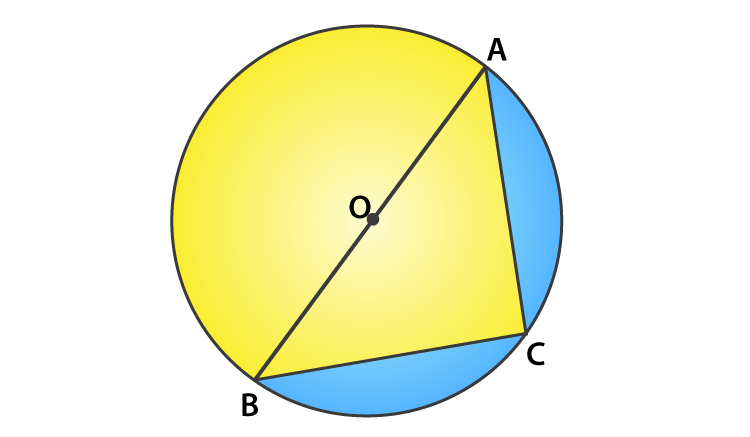Given,

AC = 24 cm and BC = 10 cm

Since, AB is the diameter of the circle

Angle ACB = 90o

So, using Pythagoras theorem

AB2 = AC2 + BC2 = 242 + 102 = 576 + 100 = 676

AB = √676 = 26 cm

Thus, the radius of the circle = 26/2 = 13 cm

The area of shaded region = Area of semi-circle – Area of triangle ACB

= ½ πr2 – ½ x b x h

= ½ (22/7)132 – ½ x 10 x 24

= 265.33 – 120

= 145.33 cm2

24. A circle is inscribed in an equilateral triangle ABC of side 12 cm, touching its sides (fig.,). Find the radius of the inscribed circle and the area of the shaded part.

Solution: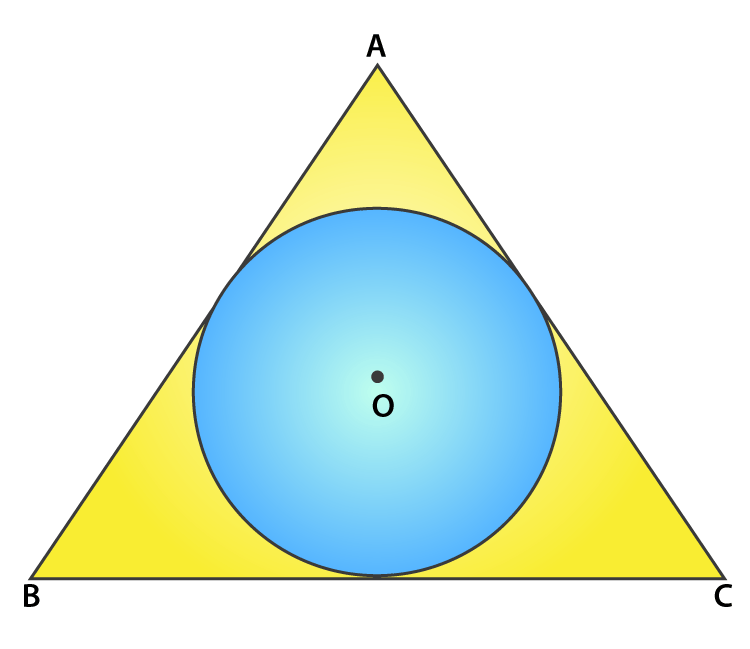Given,

An equilateral triangle of side = 12 cm

Area of the equilateral triangle = √3/4(side)2

= √3/4(12)2 = 36√3 cm2

Perimeter of triangle ABC = 3 x 12 = 36 cm

So,

The radius of incircle = Area of triangle/ ½ (perimeter of triangle)

= 36√3/ ½ x 36

= 2√3 cm

Therefore,

Area of the shaded part = Area of equilateral triangle – Area of circle

= 36√3 – πr2

= 36(1.732) – (3.14)(2√3)2

= 62.352 – 37.68

= 24.672 cm2

25. In fig., an equilateral triangle ABC of side 6 cm has been inscribed in a circle. Find the area of the shaded region. (Take π = 3.14).

Solution: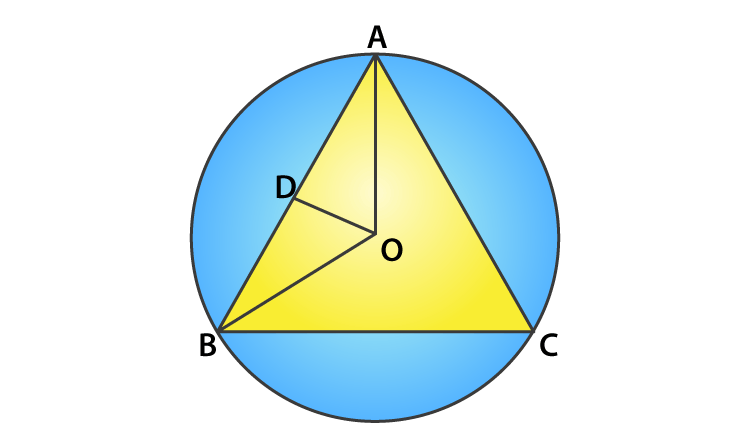Given,

Side of the equilateral triangle = 6 cm

And,

The area of the equilateral triangle = √3/4(side)2

= √3/4(6)2

= √3/4(36)

= 9√3 cm2

Let us mark the center of the circle as O, OA and OB are the radii of the circle.

In triangle BOD,

sin 60o = BD/ OB

√3/2 = 3/ OB

OB = 2√3 cm = r

Therefore,

The area of shaded region = Area of the circle – area of the equilateral triangle

= πr2 – 9√3

= 3.14 x (2√3)2 – 9√3

= 3.14 x 12 – 9 x 1.732

= 37.68 – 15.588

= 22.092 cm2

### Significance of RD Sharma Solutions are as follows:

• Practising RD Sharma Solutions in a comprehensive manner not only boosts confidence in solving the problems but also enhances their conceptual knowledge.
• RD Sharma Solutions offer the precise answers  in a simple language as per the students grasping skills.
• The solutions are developed by highly experienced teachers to help students in effective learning of concepts and also clear their doubts quickly.
• For better academic performance, students are suggested to follow the solutions PDF in both online and offline mode.

## Frequently Asked Questions on RD Sharma Solutions for Class 10 Maths Chapter 15

### Why is it important to follow RD Sharma Solutions for Class 10 Maths Chapter 15 from BYJU’S?

Experts at BYJU’S provide 100% precise answers to help students to obtain in-depth knowledge of concepts. The solutions are formulated after doing huge research on each topic. Students who get stuck while solving the textbook problems are recommended to use solutions PDF and get their doubts cleared. The simple and accurate answers in an understandable language enhance a student’s problem solving skills which is essential from an exam point of view.

### How does RD Sharma Solutions for Class 10 Maths Chapter 15 help students to clear their doubts immediately?

RD Sharma Solutions for Class 10 Maths Chapter 15 offered by BYJU’S is comprehensive based on the latest guidelines set by the CBSE board. The concepts are designed in a simple and lucid manner to improve skills in solving complex problems in an efficient manner. RD Sharma Solutions are prepared in such a way that students clear their doubts quickly and learn the concepts more effectively.

### How should I boost the exam preparation using the RD Sharma Solutions for Class 10 Maths Chapter 15?

Highly experienced teachers advise students to choose the correct study source for effective exam preparation. RD Sharma Solutions for Class 10 Maths Chapter 15 provide detailed explanation along with pictorial representation for better understanding of concepts among students. Practising the solution module on a daily basis helps students to get a stronghold on the covered concepts. The precise solutions prepared by expert tutors boost interest in learning the concepts thoroughly.

1. Nandana

Good one 👍